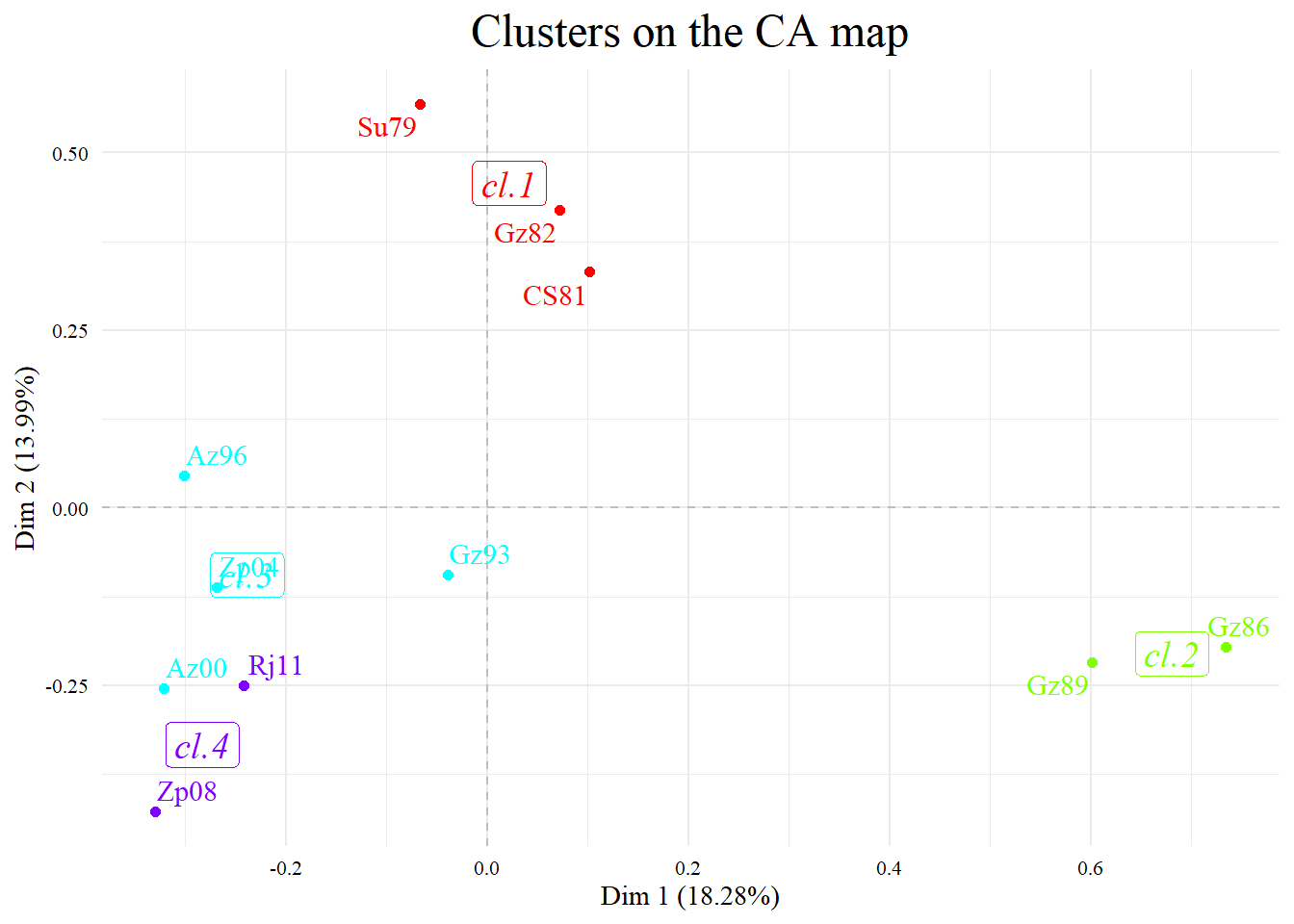# Spanish Discourses _Pg5

## 5. Plotting maps with plot.LexHCca. Documents

### Previous syntax

###### load(url("http://www.xplortext.org/Rdata/SpanishDisc.RData")) library(Xplortext) swu <- c("consiguiente", "ello", "hacia", "punto", "Señorías", "si", "Sus", "vista", "A", "B", "C", "D", "E", "F", "a", "b", "c", "d")

To build a new TextDataObject with a minimum of 10 frequency of words and at least in 2 documents:

###### TD <- TextData(SpanishDisc, var.text=c(1), context.quanti="year", Fmin=10, Dmin=2, idiom="es", lower=FALSE, remov.number=TRUE, stop.word.tm=TRUE, stop.word.user=swu, graph=FALSE)

To select only the first 3 factors and 4 cluster without contiguity restriction:

### 5.1. Selecting clusters and cases

#### Selecting clusters 1 and 4

###### plot(res.HCca, type="map", plot=c("points", "labels", "centers"), selClust=c(1,4))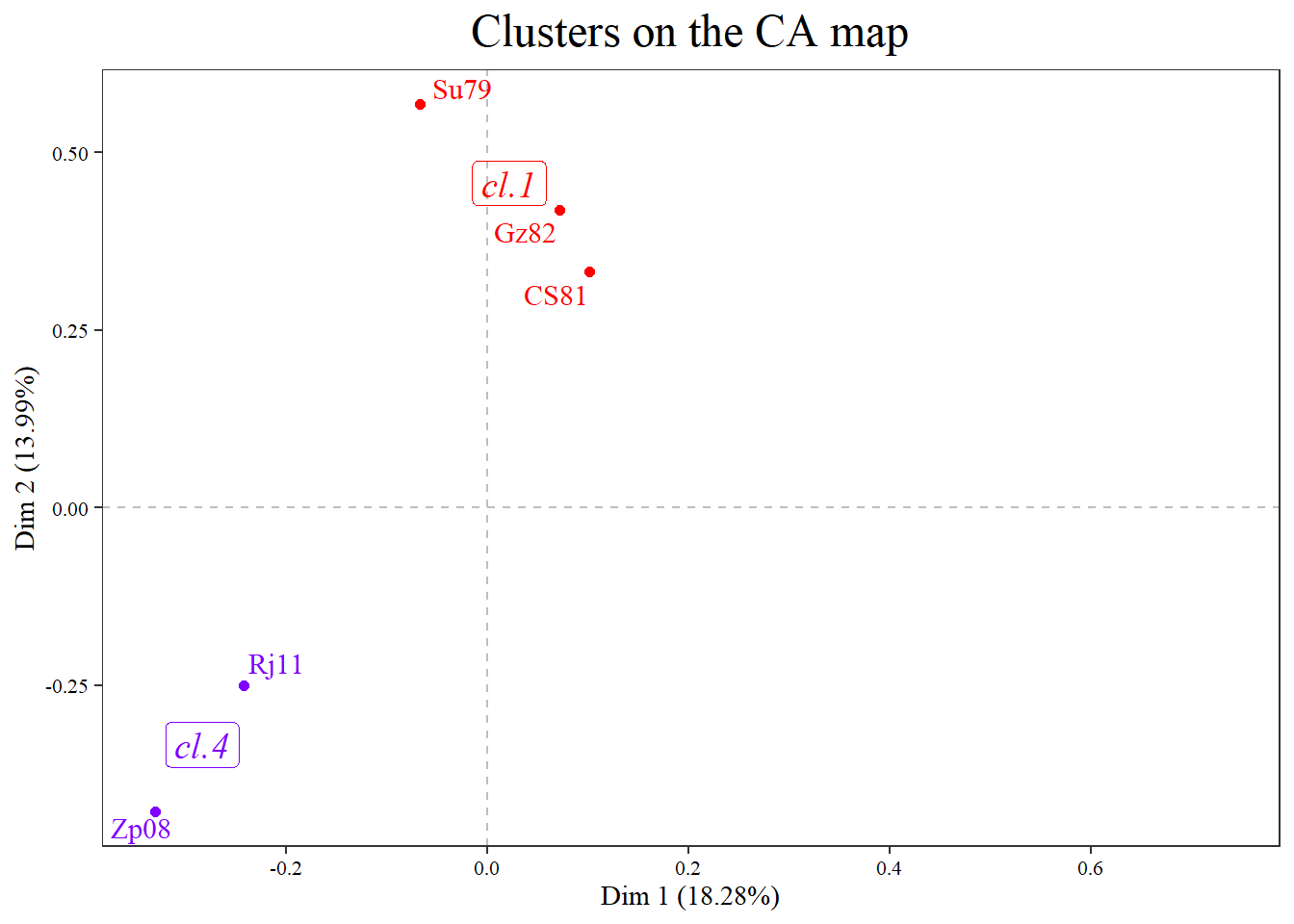selClust: vector indexes with the number of the clusters to plot (by default “ALL”)

selInd: vector with the active documents/words to plot (indexes, names or rules; see details; by default “ALL”)

###### plot(res.HCca, type="map", plot=c("labels", "centers"), selClust=c(1,4))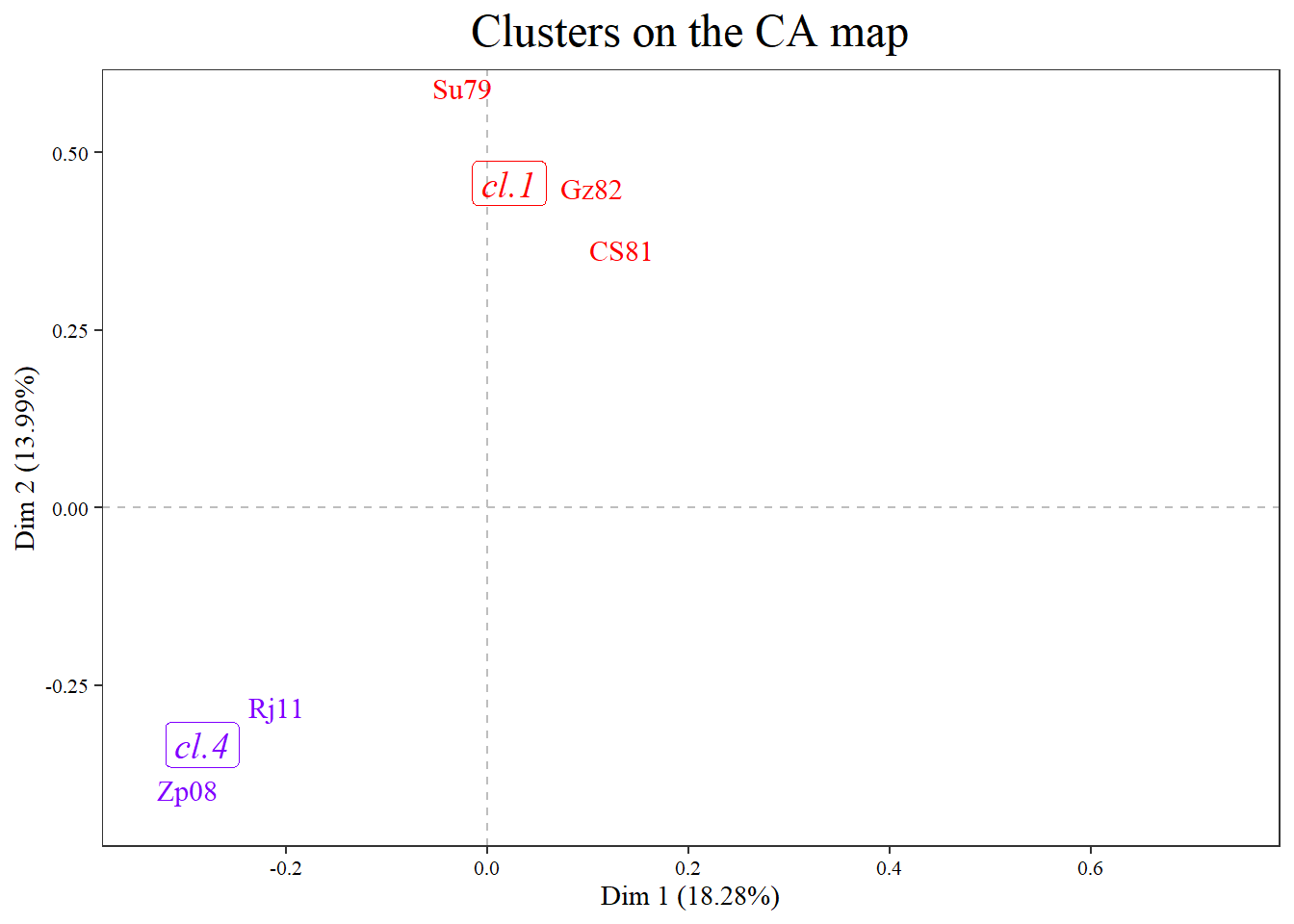Selecting all the cases of cluster 1 and the center of cluster 4 but not cases of cluster 4

###### plot(res.HCca, type="map", plot=c("labels", "centers"), selClust=c(1,4), selInd=c(1:3))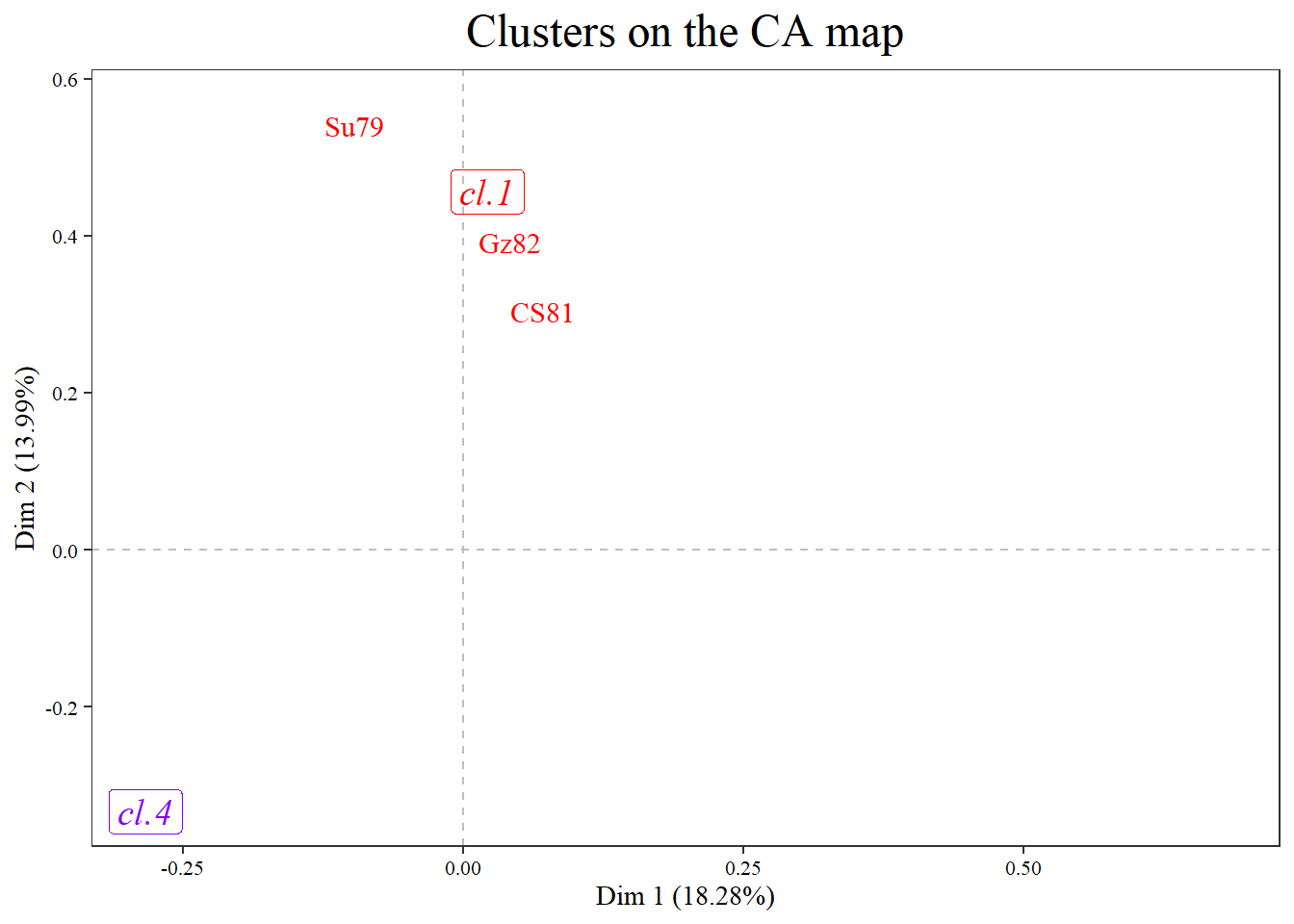Selecting some cases by name and all the centers

###### plot(res.HCca, type="map", plot=c("labels", "centers"), selInd=c("Su79", "CS81", "Rj11"))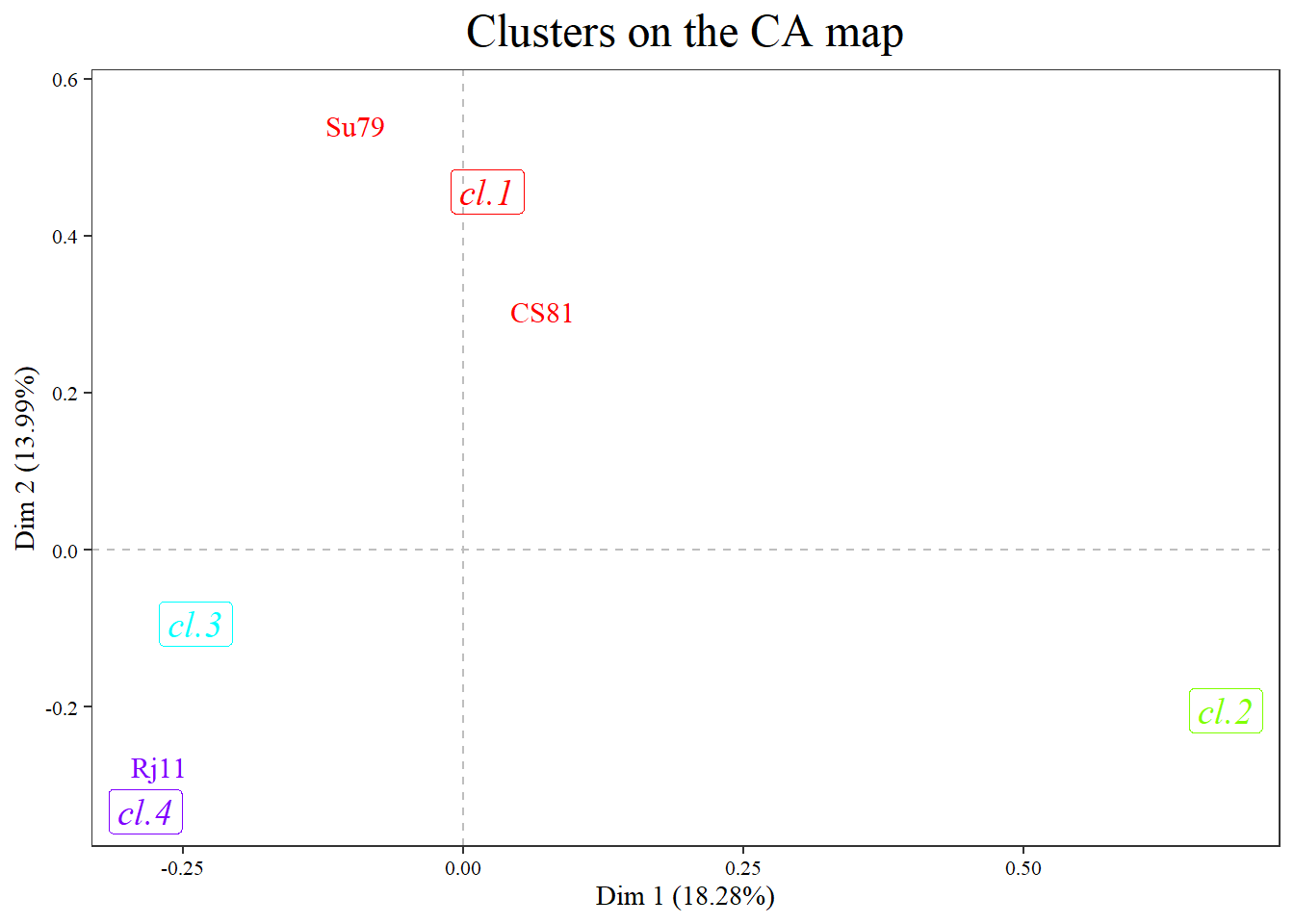Selecting only the 5 cases with the highest coordinates.

###### plot(res.HCca, type="map", plot=c("labels", "centers"), selInd="coord 5")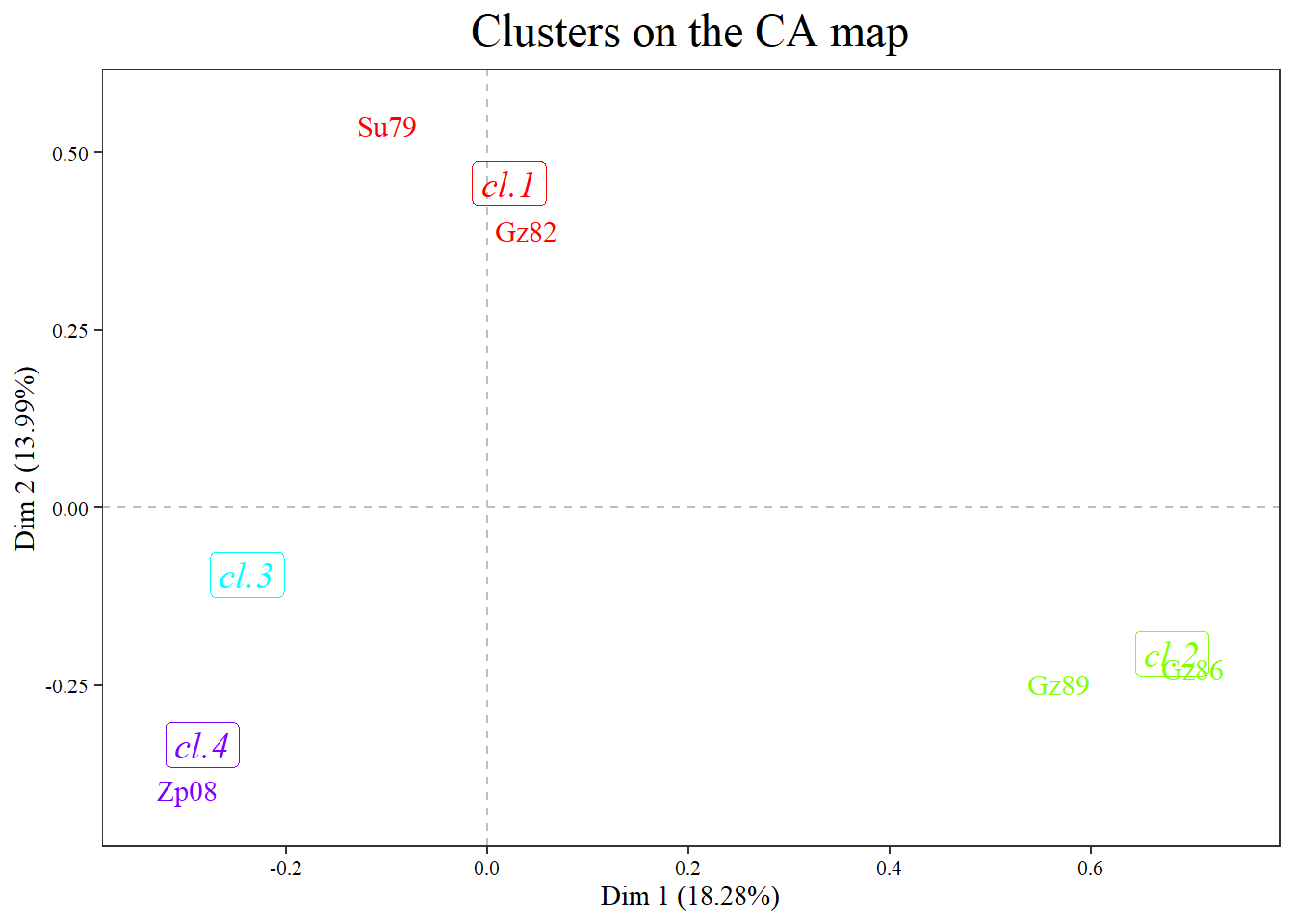Selecting only the cases with a contribution to the inertia, of any of the 2 axes over 16 percent.

###### plot(res.HCca, type="map", plot=c("labels", "centers"), selInd="contrib 16")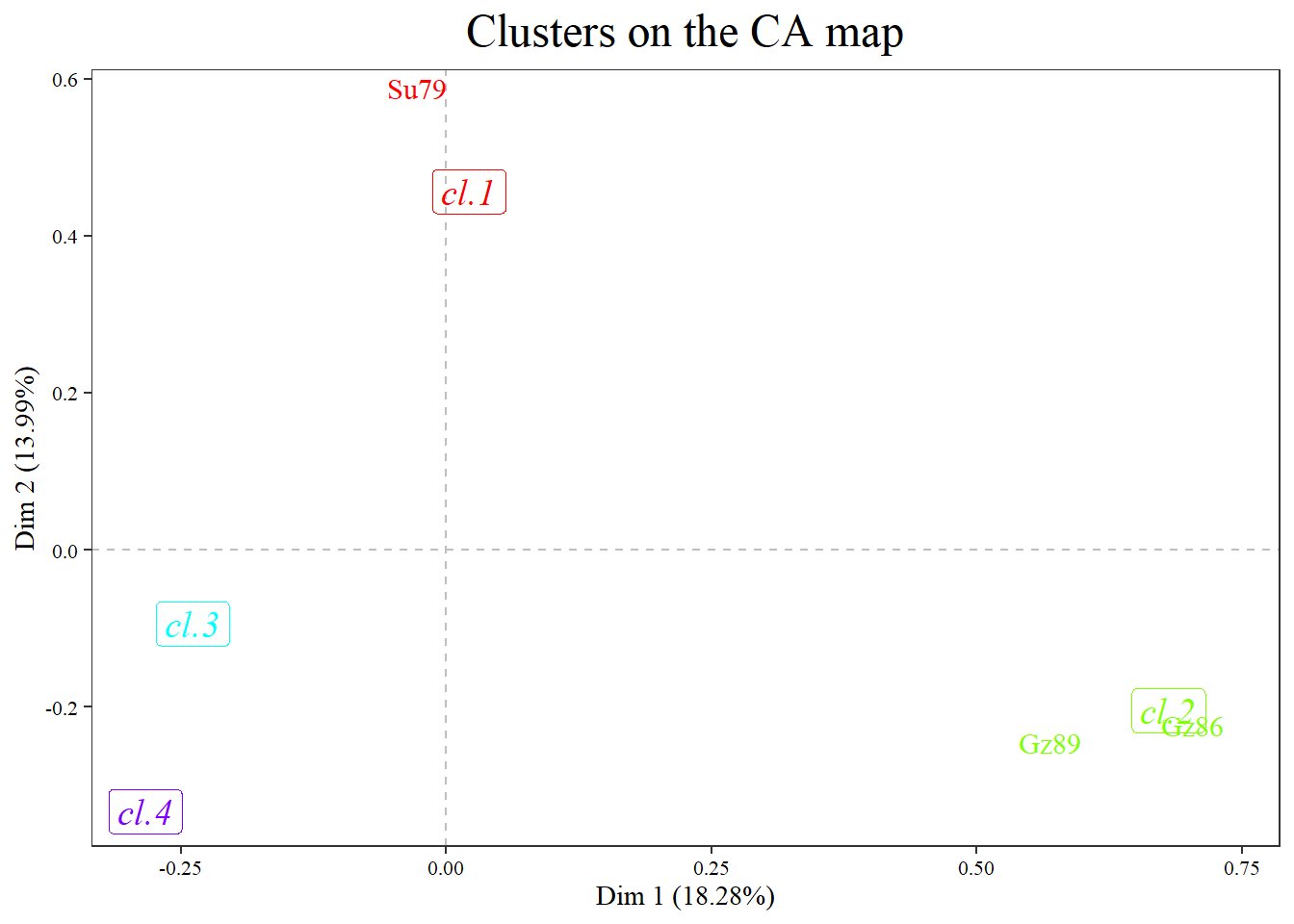The documents with a cos2 over 0.50, as summed on the 2 axes, are plotted.

###### plot(res.HCca, type="map", plot=c("labels", "centers"), selInd="cos2 .50")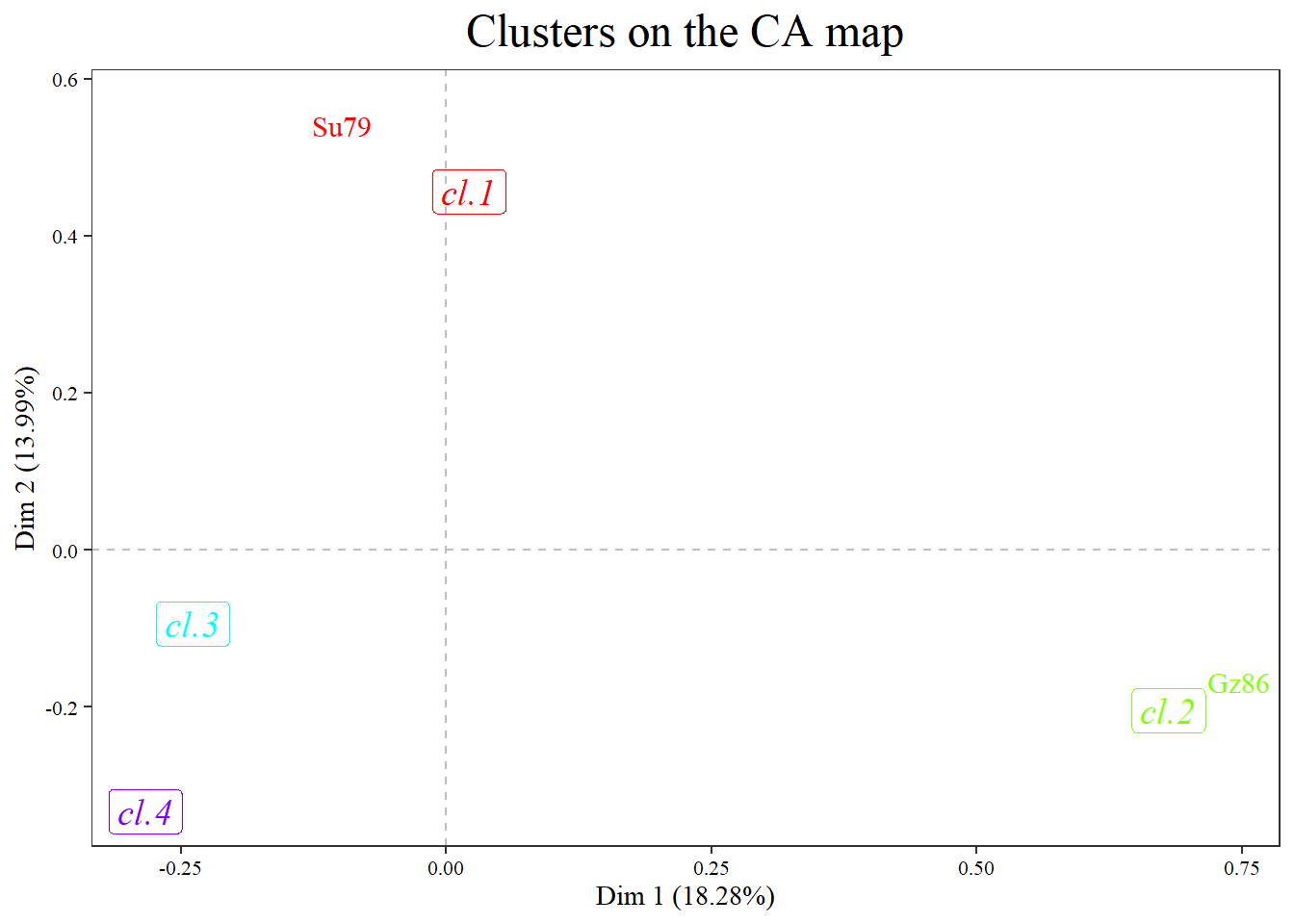selInd=“meta 3”: the cases with a contribution over 1 times the average word/document contribution on any of the two axes are plotted.

###### plot(res.HCca, type="map", plot=c("labels", "centers"), selInd="meta 1")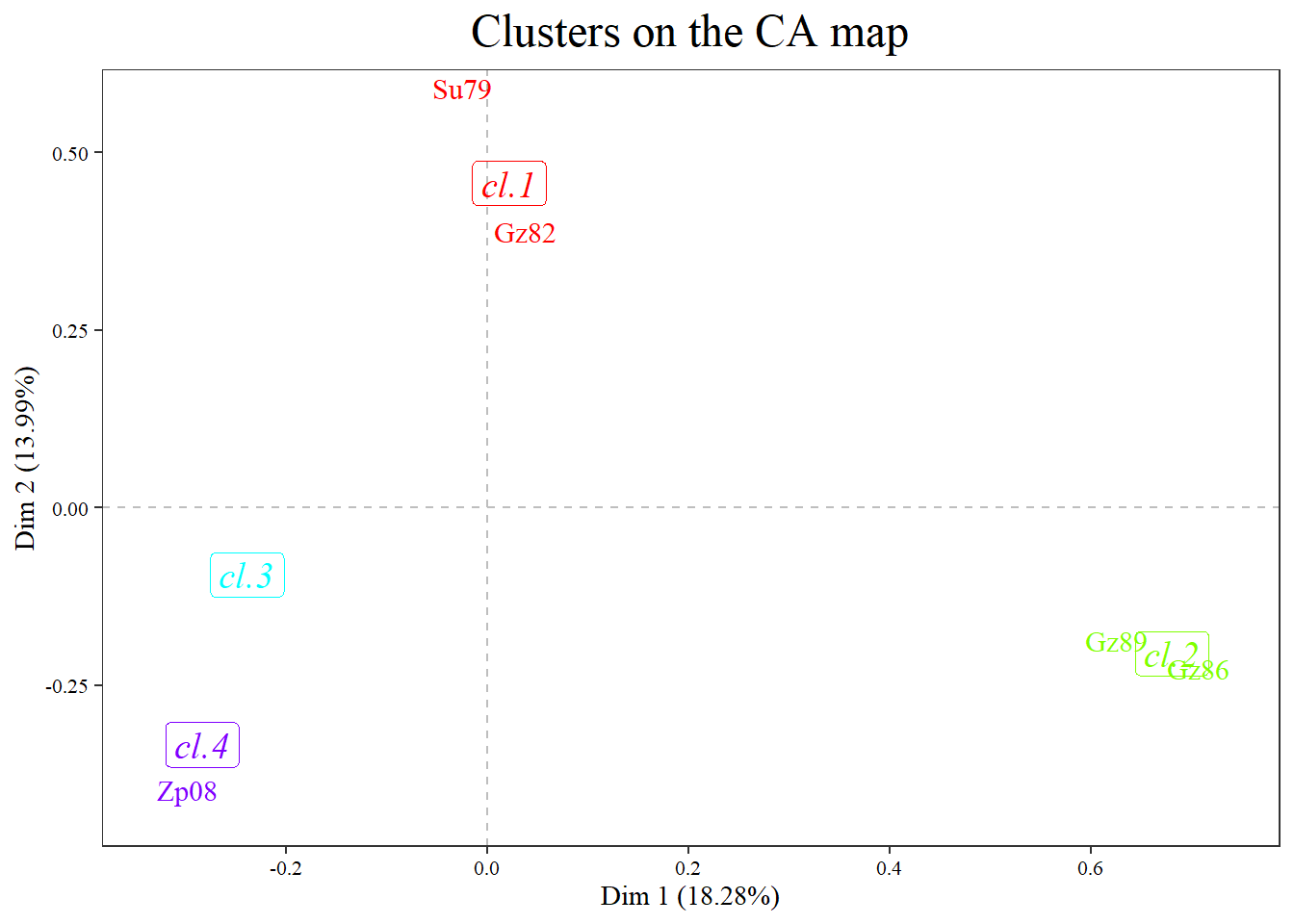### 5.2. Add trajectory to cases

Draws trajectory arrows in the order of clusters or in the selInd order.

Parameters:

color (by default blue),

linetype (by default 1 solid),

space (by default 0 and no space is added from point to arrow, be carefull with this value)

size (width,by default 1),

arrow.length (of the arrow, by default .3),

arrow.type (by default “closed”),

arrow.angle (by default 30),

alpha.t (transparency from 0 invisibility to 1 opaque) (by default 1)

See geom_segment for details.

###### plot(res.HCca, type="map", plot=c("points", "labels", "centers", "traject"), traject=c(color="orange", linetype="dotted"))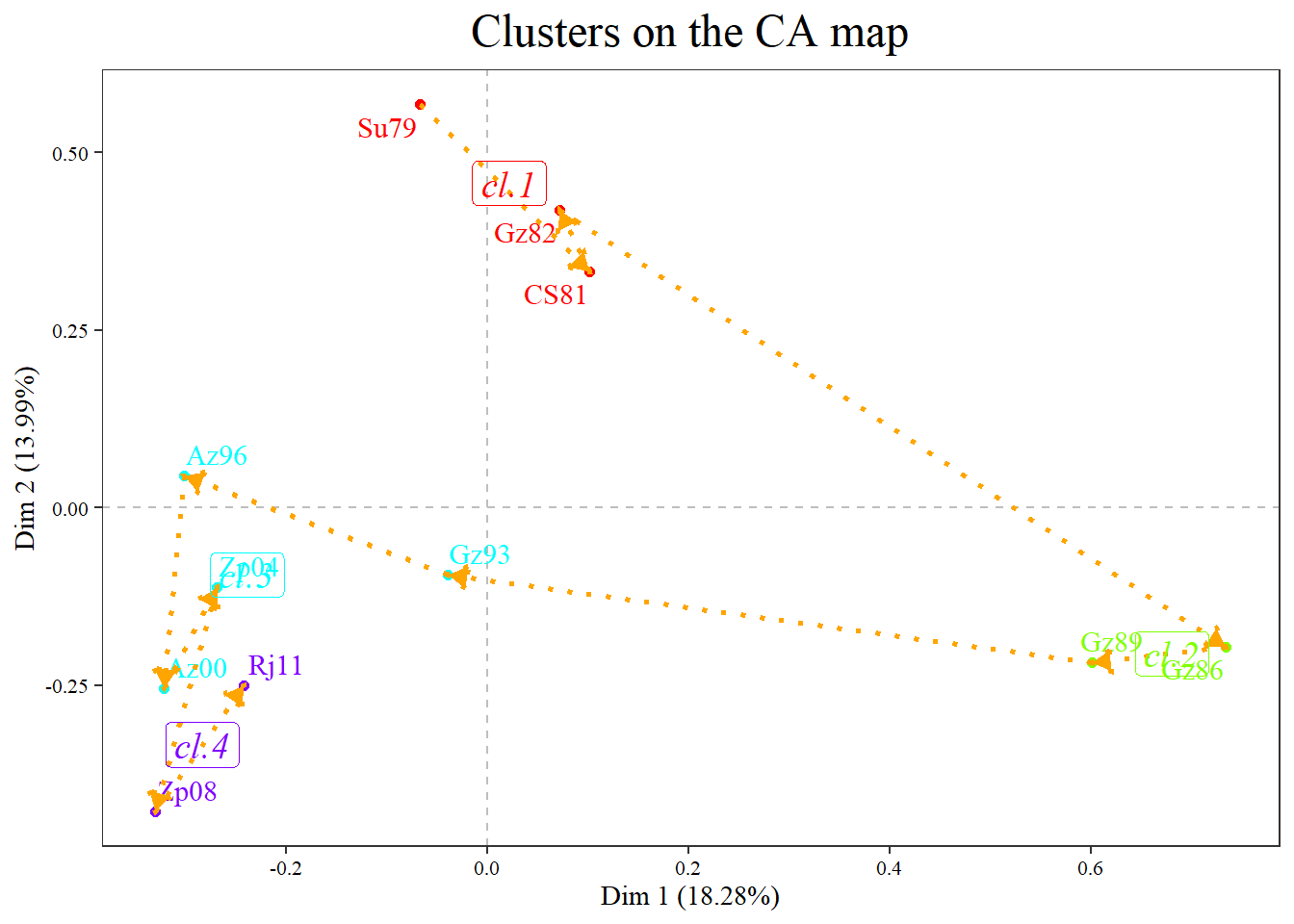###### plot.LexHCca(res.HCca, selInd=c(1,2,3,8,9), plot=c("points", "traject","labels", "centers"))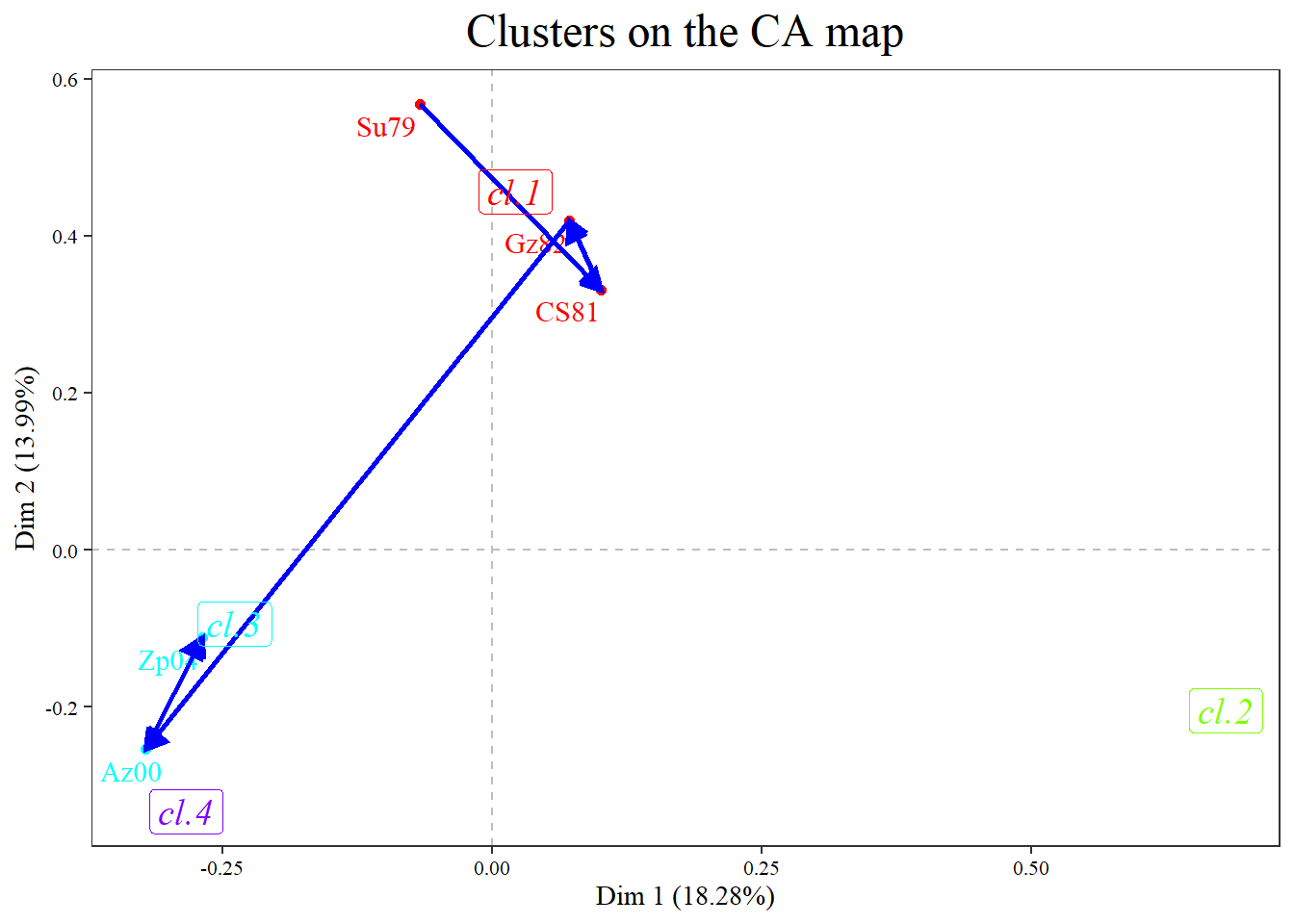Draws a hull with all the cases of each cluster.

Parameters:

type (ellipse, by default, hull),

alpha.t (transparency from 0 invisibility to 1 opaque) (by default .1)

color, linetype (by default “dotted”))

###### plot(res.HCca, type="map", plot=c("labels", "centers", "hull"))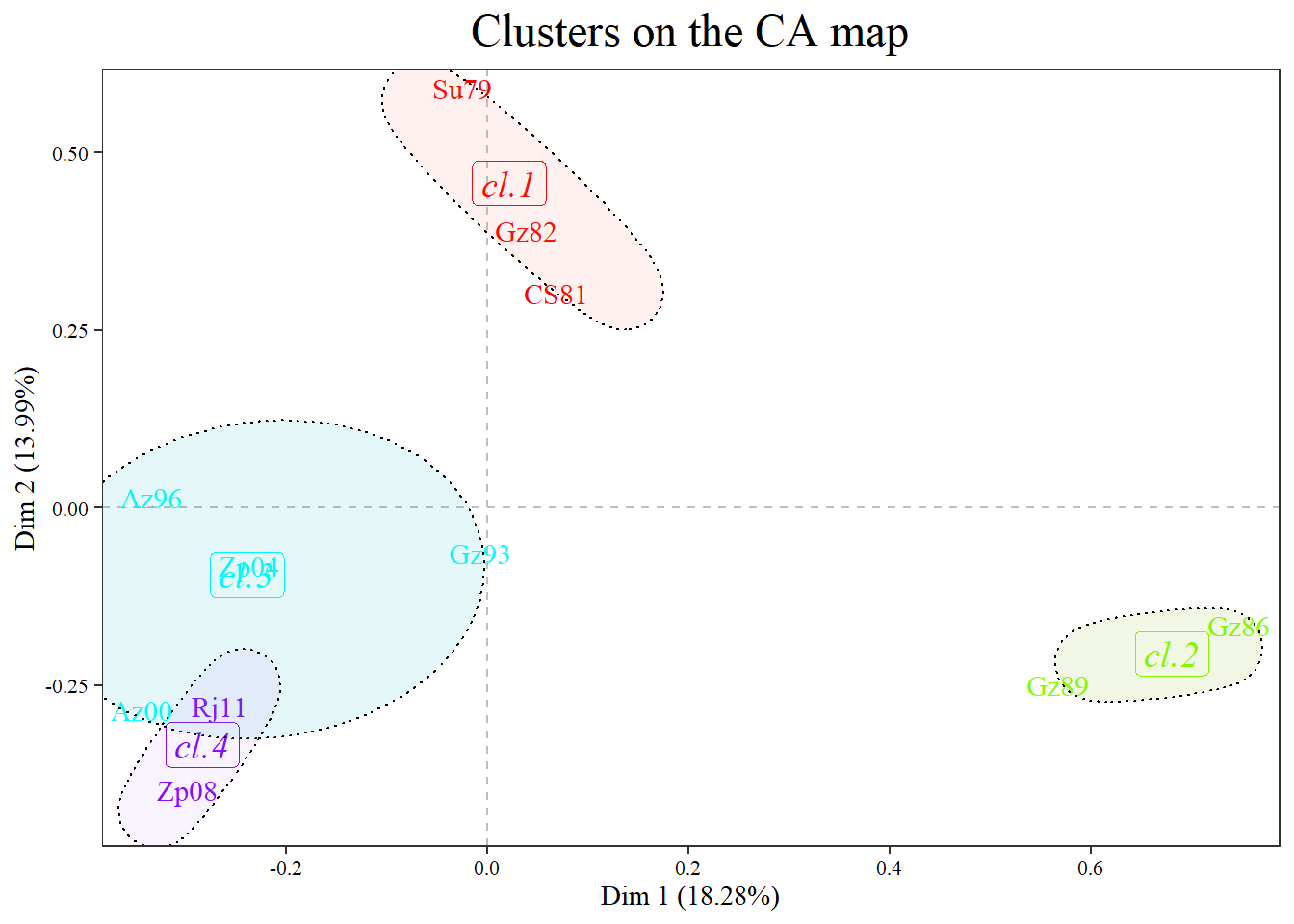###### plot(res.HCca, type="map", plot=c("labels", "centers", "hull"), hull=c(type="hull"),ylim=c(-.5,.75),)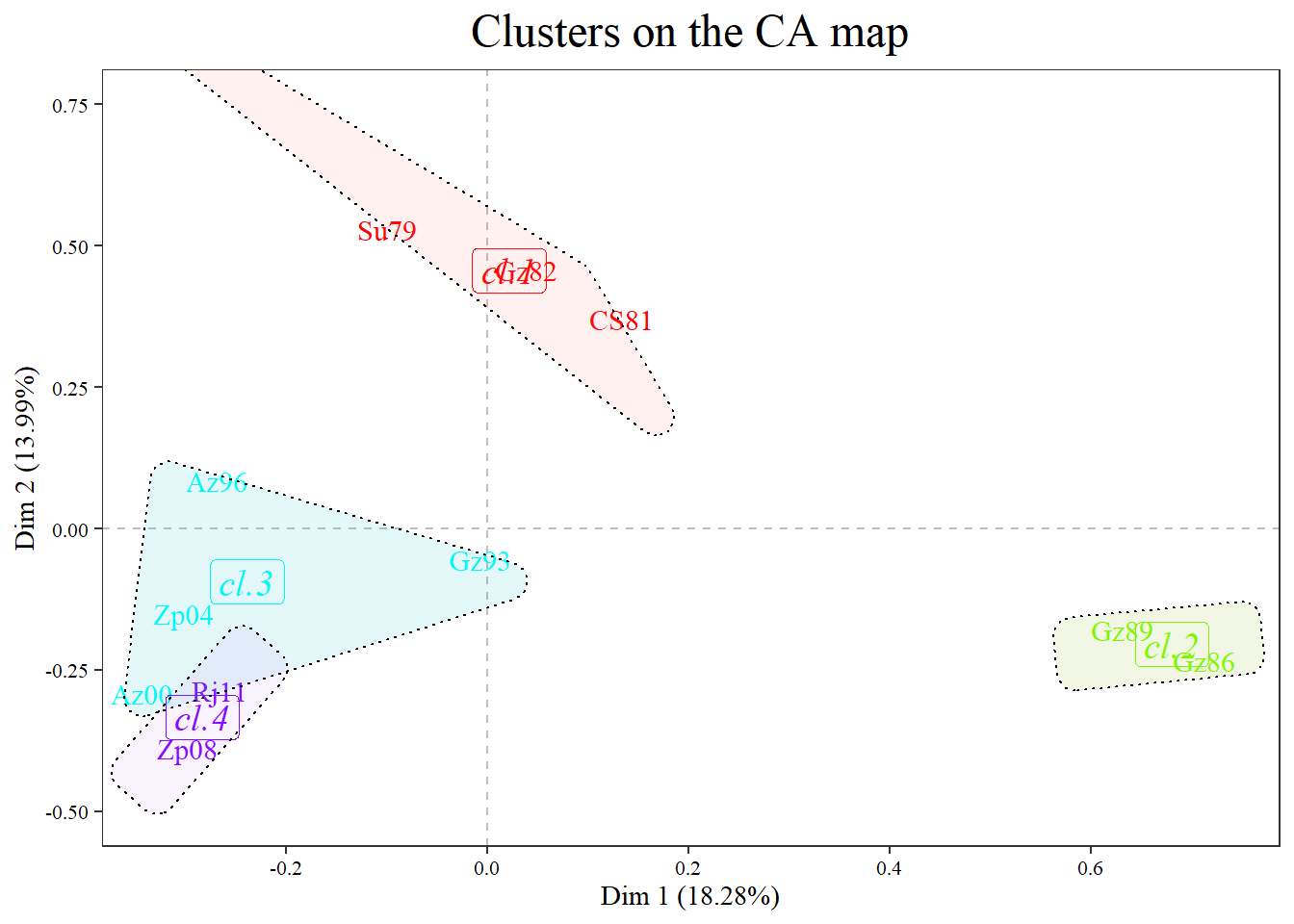Changing colors of hull and transparency

###### plot(res.HCca, type="map", plot=c("labels", "centers", "hull"), hull=c(type="hull", color="blue", alpha.t=0), ylim=c(-.5,.75))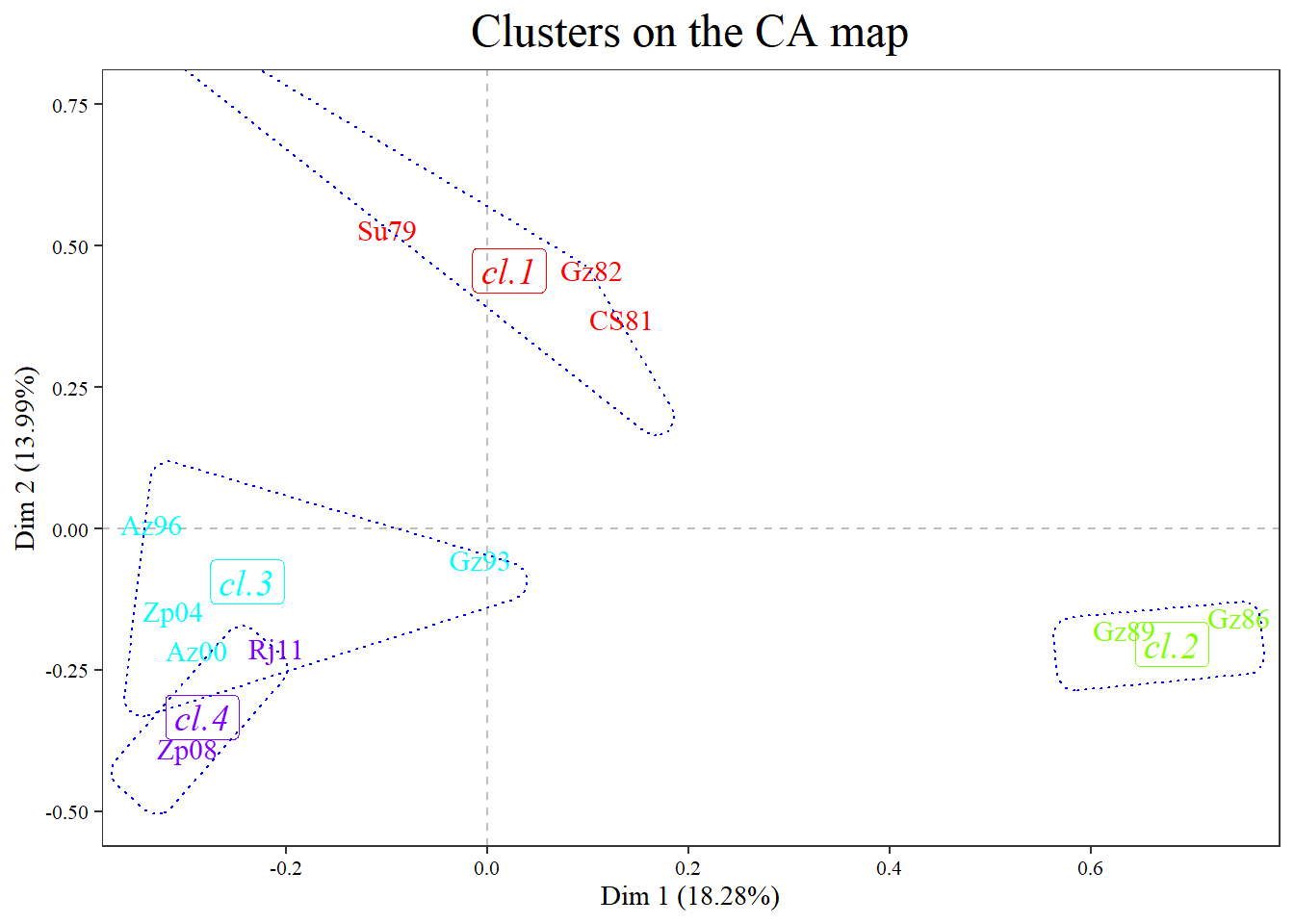### 5.4. Changing palette

palette, the color of the palette used to draw the points. By default colors are chosen. If you want to define the colors for 4 clusters :

###### plot(res.HCca, type="map", plot=c("labels", "centers"), palette=c("brown","red","blue","forestgreen"))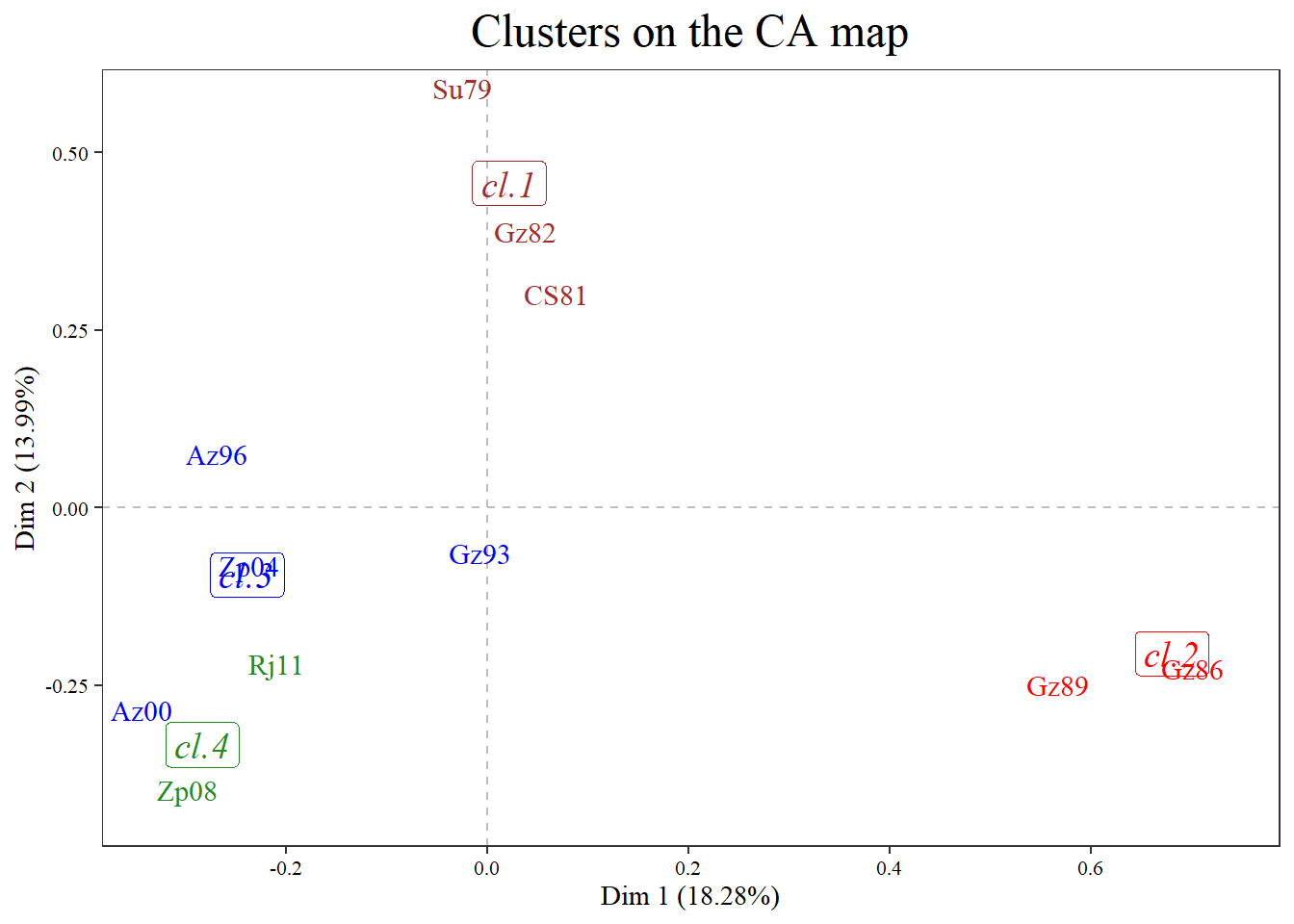### 5.5. Format of points

Plot only points

###### plot(res.HCca , type="map", plot="points")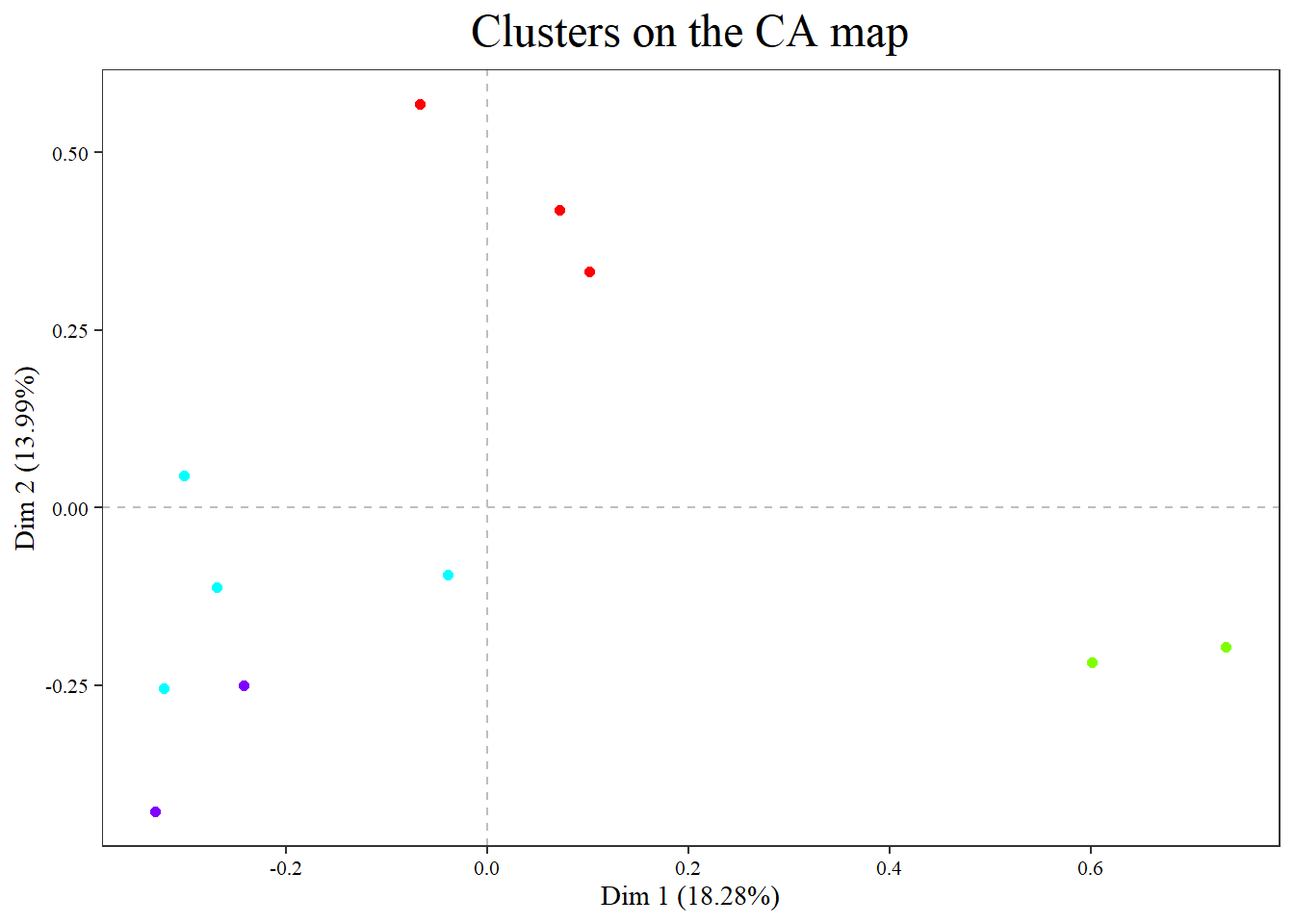format of points. Parameters:

size (if size=0 points are no plotted),

shape (by default 21),

fill (if a color, the same for all the points,

if color is NULL palette colors from clusters are applied;

if more than one color use palette argument;

only for shapes from 21 to 25 to fill the point),

stroke (controls the edge of the point (by default 0 no edge),

border (color of the border, same specifictions than fill),

alpha.t (transparency from 0 invisibility to 1 opaque) (by default 1)

See geom_point() in ggplot2 library. See details.

pch = 0,square

pch = 1,circle

pch = 2,triangle point up

pch = 3,plus

pch = 4,cross

pch = 5,diamond

pch = 6,triangle point down

pch = 7,square cross

pch = 8,star

pch = 9,diamond plus

pch = 10,circle plus

pch = 11,triangles up and down

pch = 12,square plus

pch = 13,circle cross

pch = 14,square and triangle down

pch = 15, filled square

pch = 16, filled circle

pch = 17, filled triangle point-up

pch = 18, filled diamond

pch = 19, solid circle

pch = 20,bullet (smaller circle)

pch = 21, filled circle blue

pch = 22, filled square blue

pch = 23, filled diamond blue

pch = 24, filled triangle point-up blue

pch = 25, filled triangle point down blue

Changing points:

###### plot(res.HCca , type="map", plot="points", points=c(shape=23, size=5))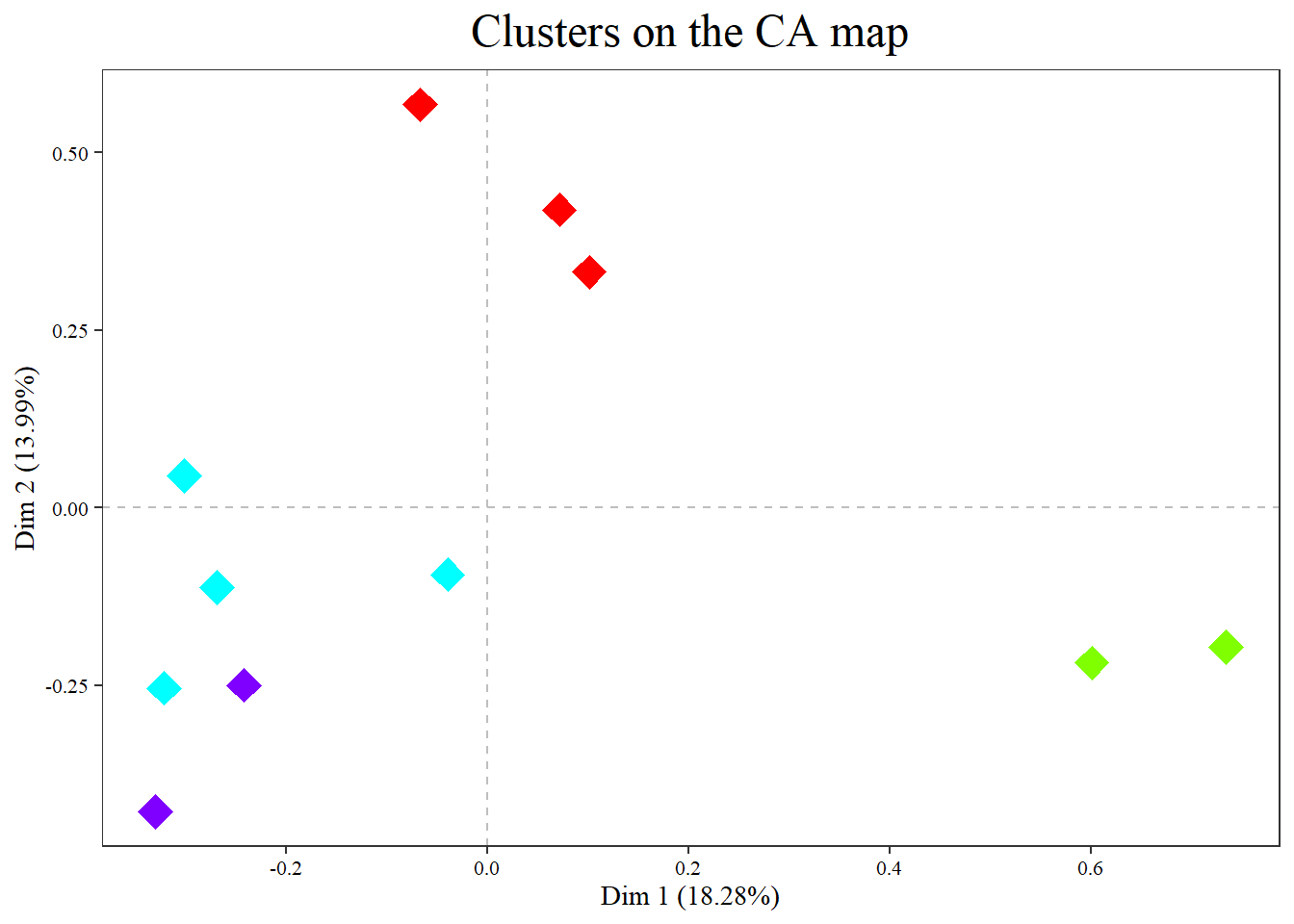###### plot(res.HCca , type="map", plot="points", points=c(shape=21, fill="black"))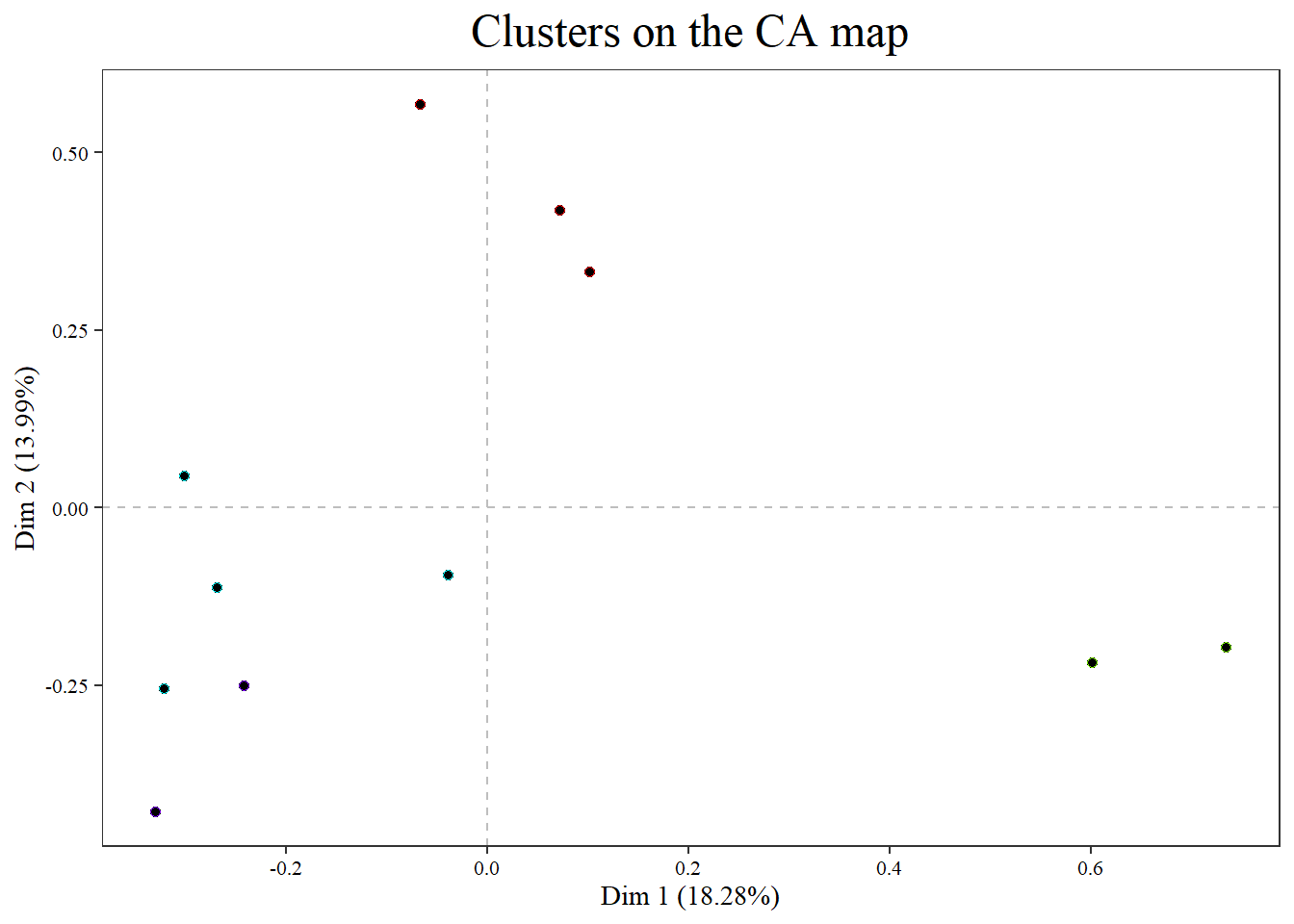###### plot(res.HCca , type="map", plot="points", points=c(shape=21, size=3, border="blue"))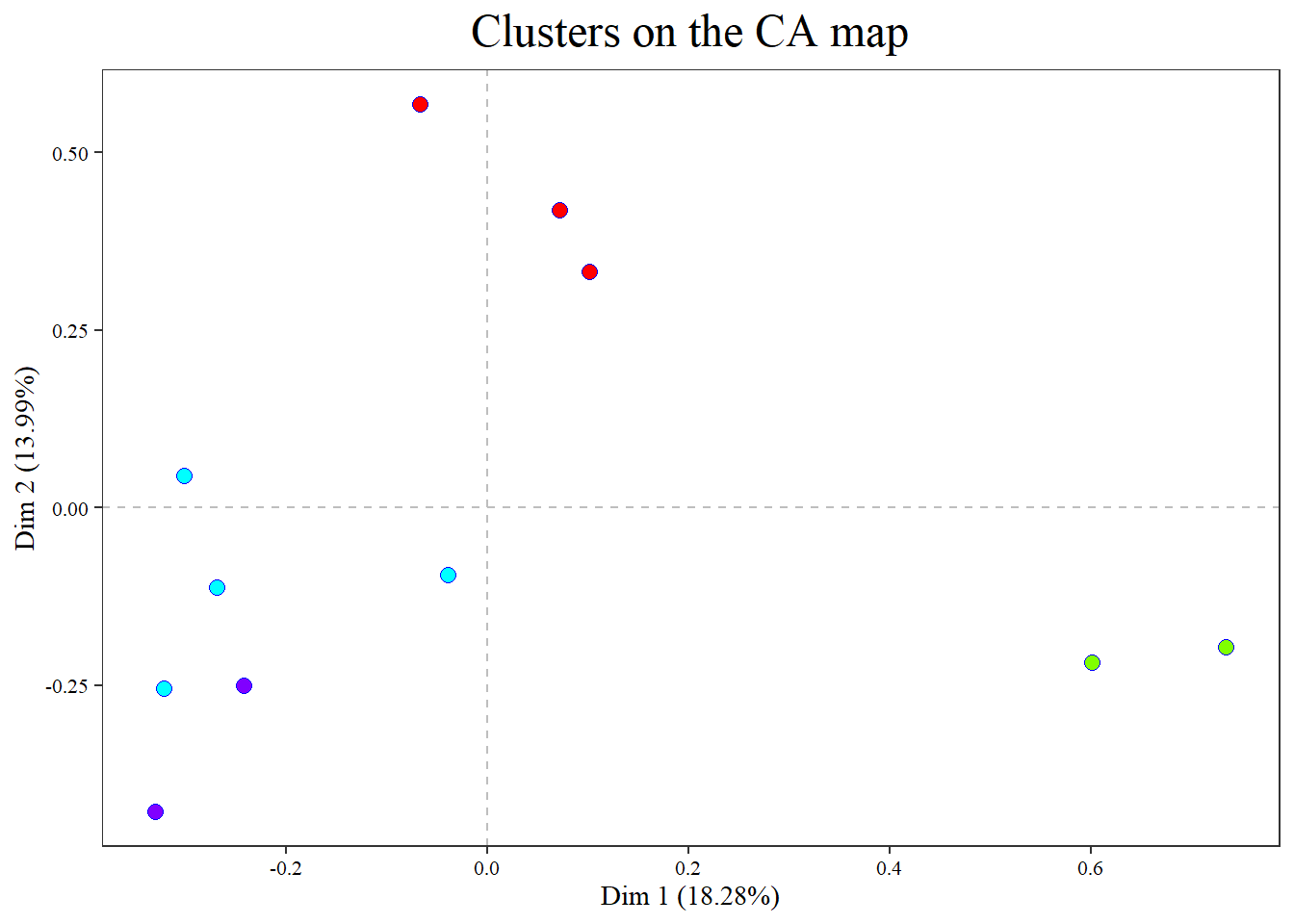###### plot(res.HCca , type="map", plot="points", points=c(shape=1, size=3, border="blue"))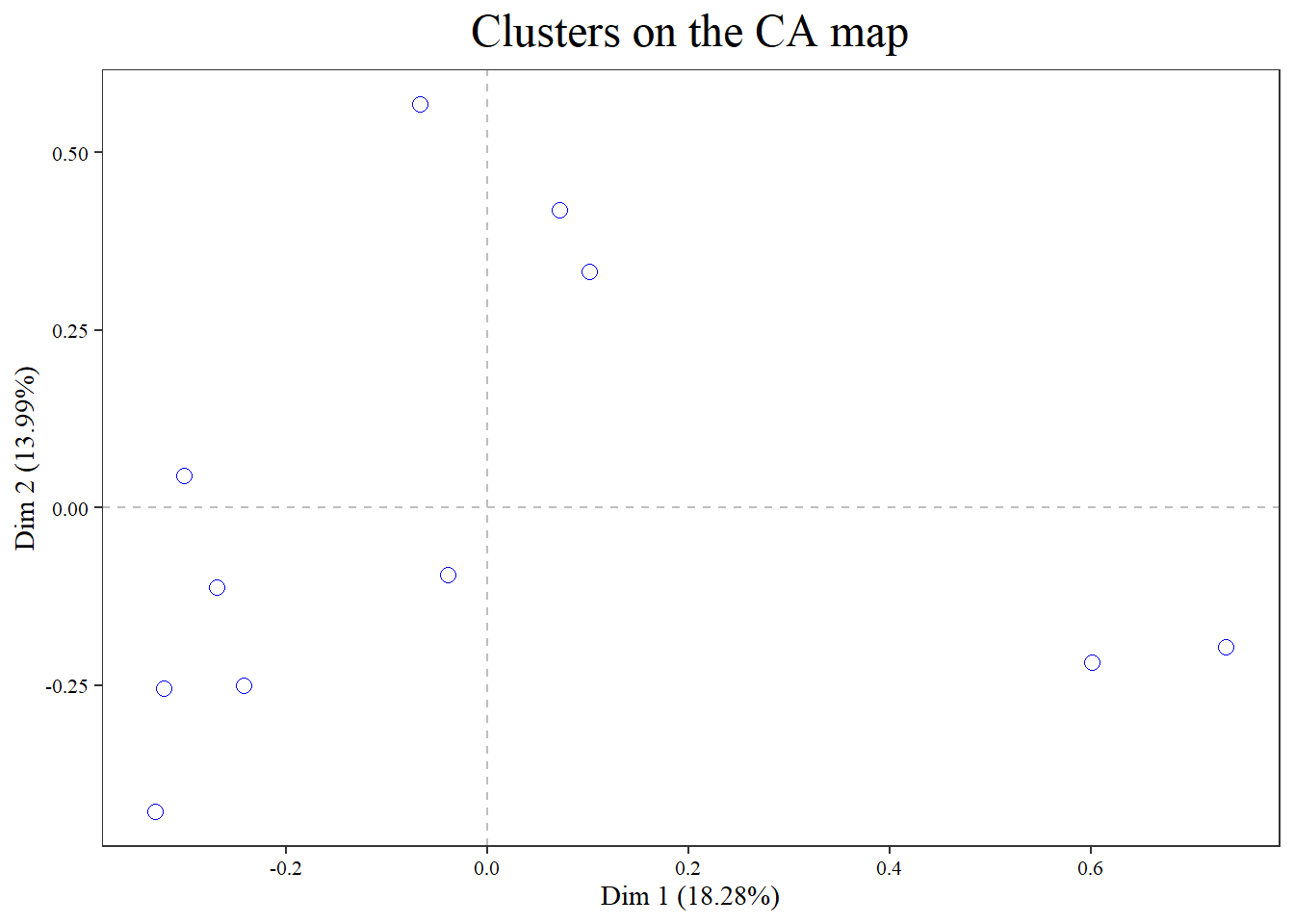###### plot(res.HCca , type="map", plot="points", points=c(shape=21, size=4, border="blue", stroke=2, alpha.t=1))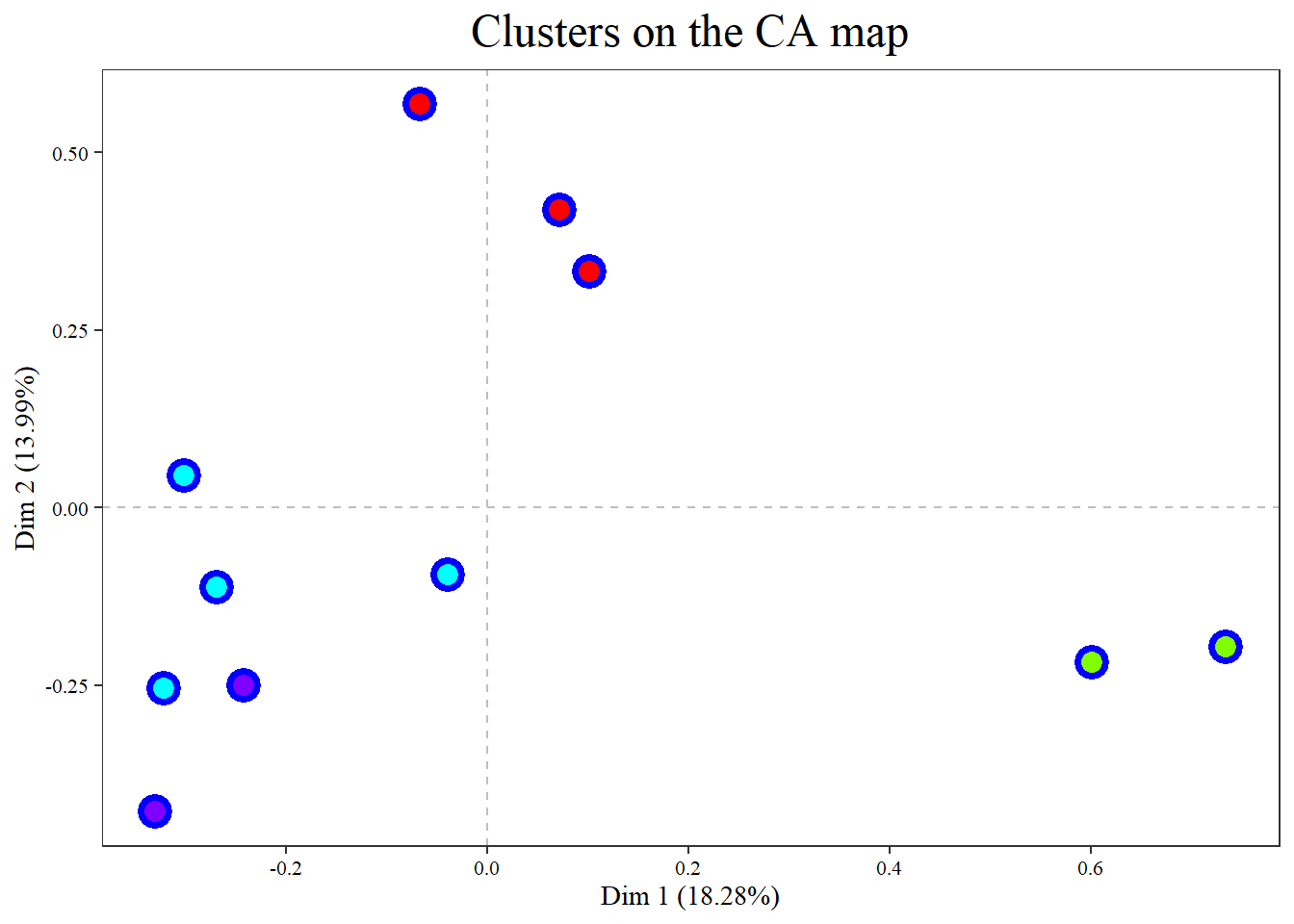###### plot(res.HCca , type="map", plot="points", points=c(shape=21, size=4, border="blue", stroke=2,  alpha.t=.2))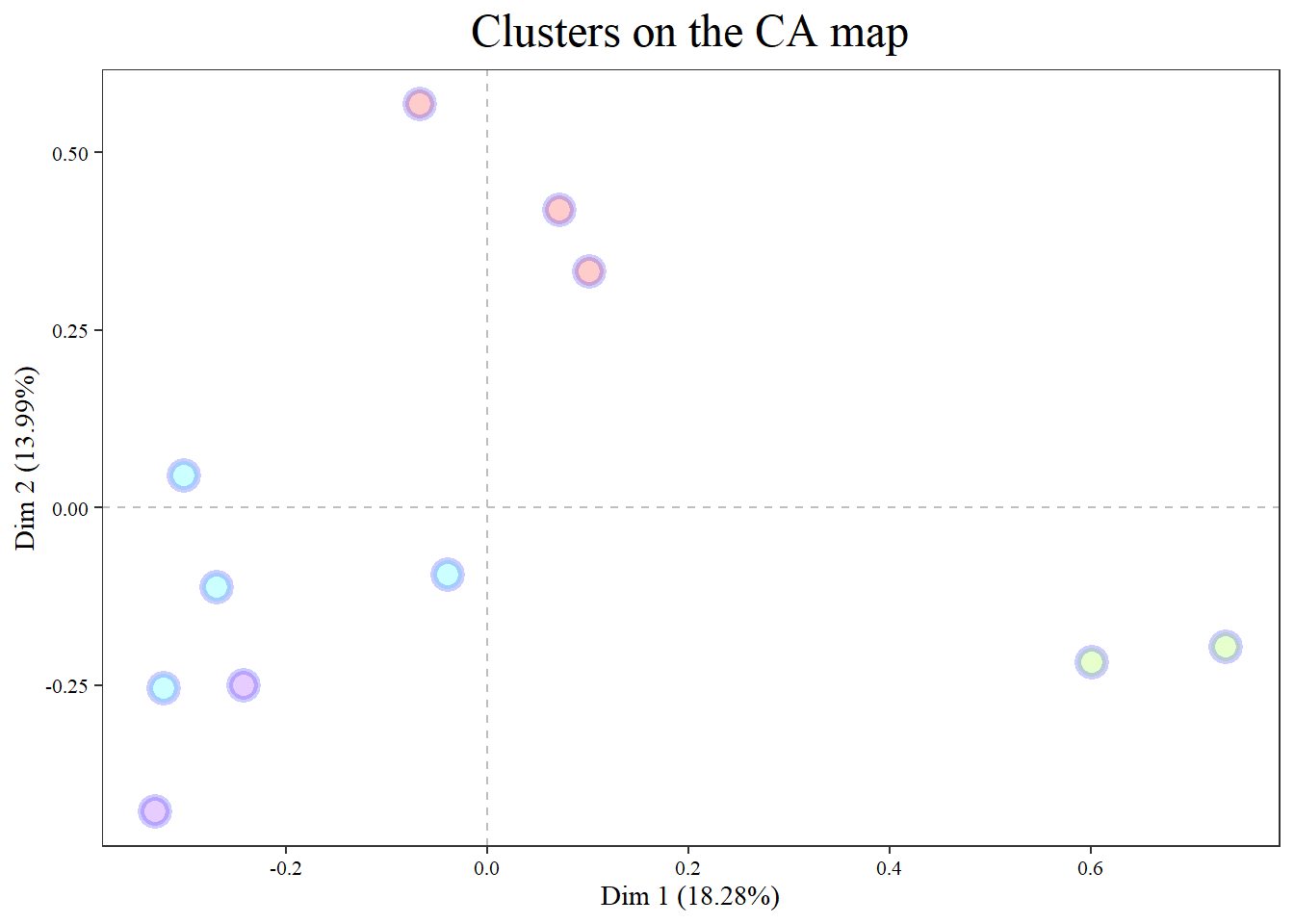### 5.6. Format of labels

format of labels. Parameters:

size (if size=0 labels are no plotted; by default 4),

family,

face,

hjust,

vjust,

color.text,

alpha.t.text,

numbers(if TRUE the label will be replaced by the number of the cluster to which it belongs, by default FALSE),

rect (if TRUE a rectangle will be drawn around the label, by default FALSE),

color.fill (color into the rectangle, by default FALSE is transparent),

alpha.t.fill, repel (to do repulsive textual annotations and make it easier to read),

set.seed (by default a new seed for each plot draws different positions, for the same seed i.e: set.seed=1234)

###### plot(res.HCca , type="map", plot="labels")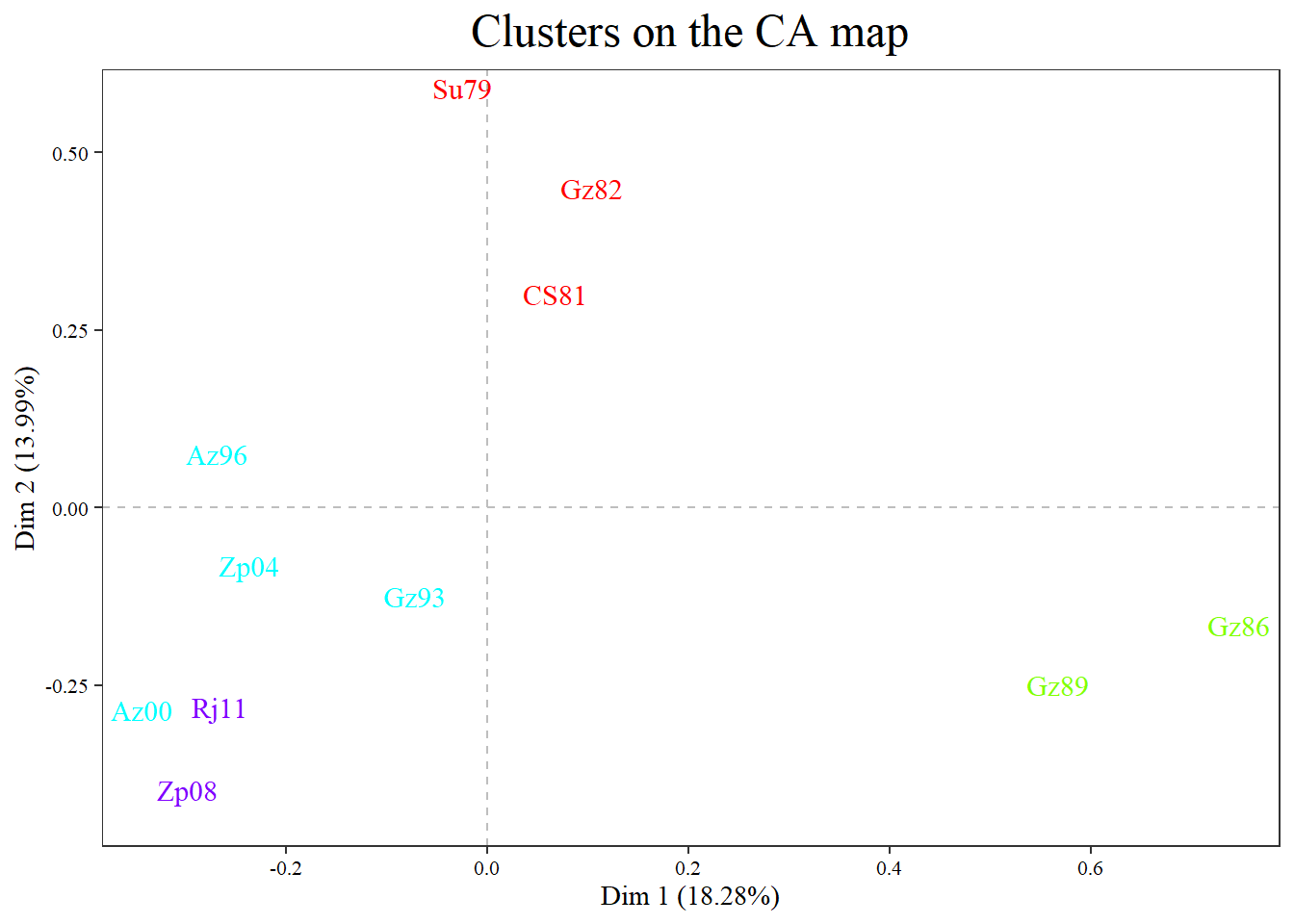force=1 (degree of repel to overlap text labels; 1 is the default)

###### plot(res.HCca , type="map", plot=c("points","labels"), labels=c(size=6, force=1))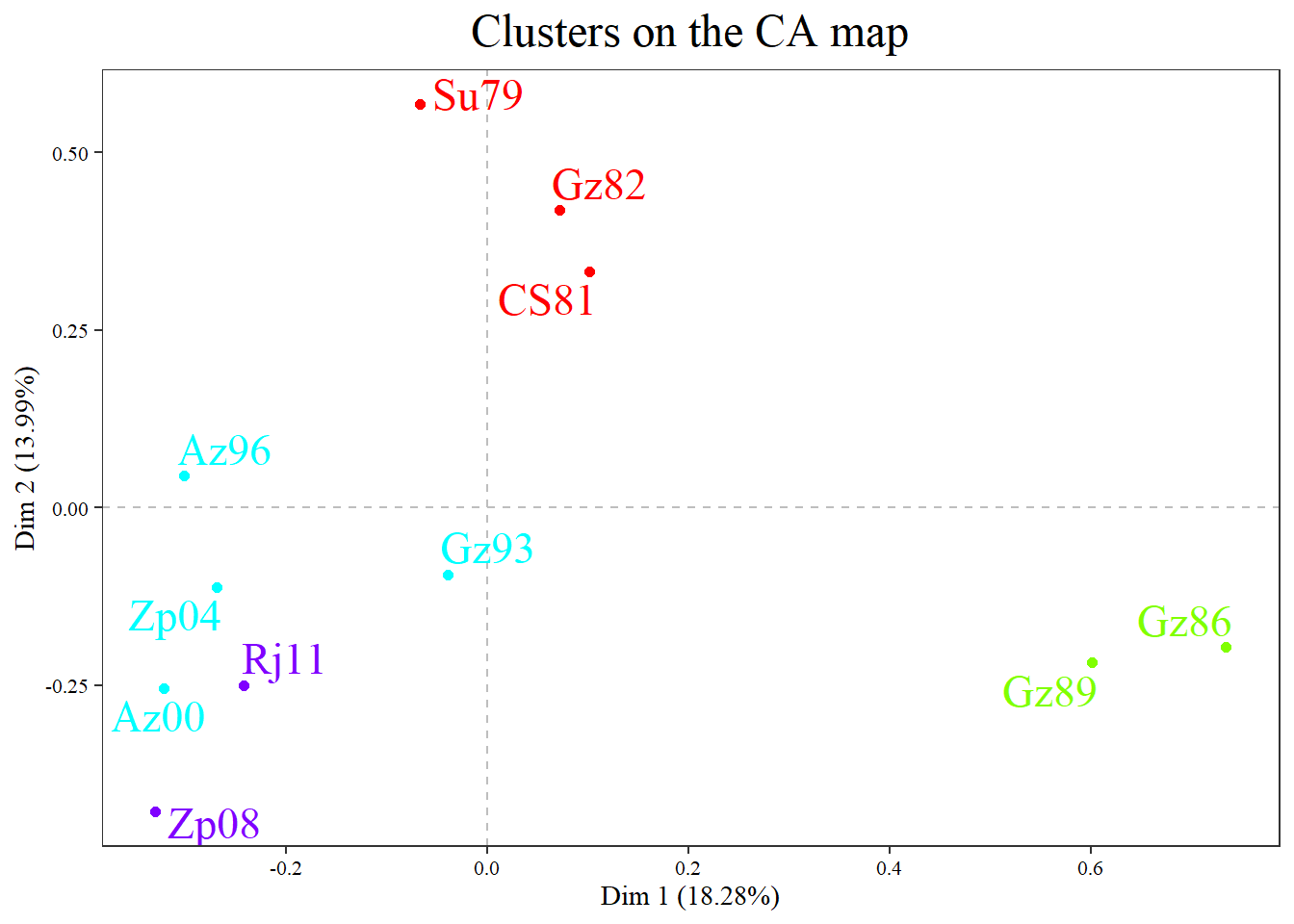###### plot(res.HCca , type="map", plot=c("points","labels"), labels=c(size=6, force=0))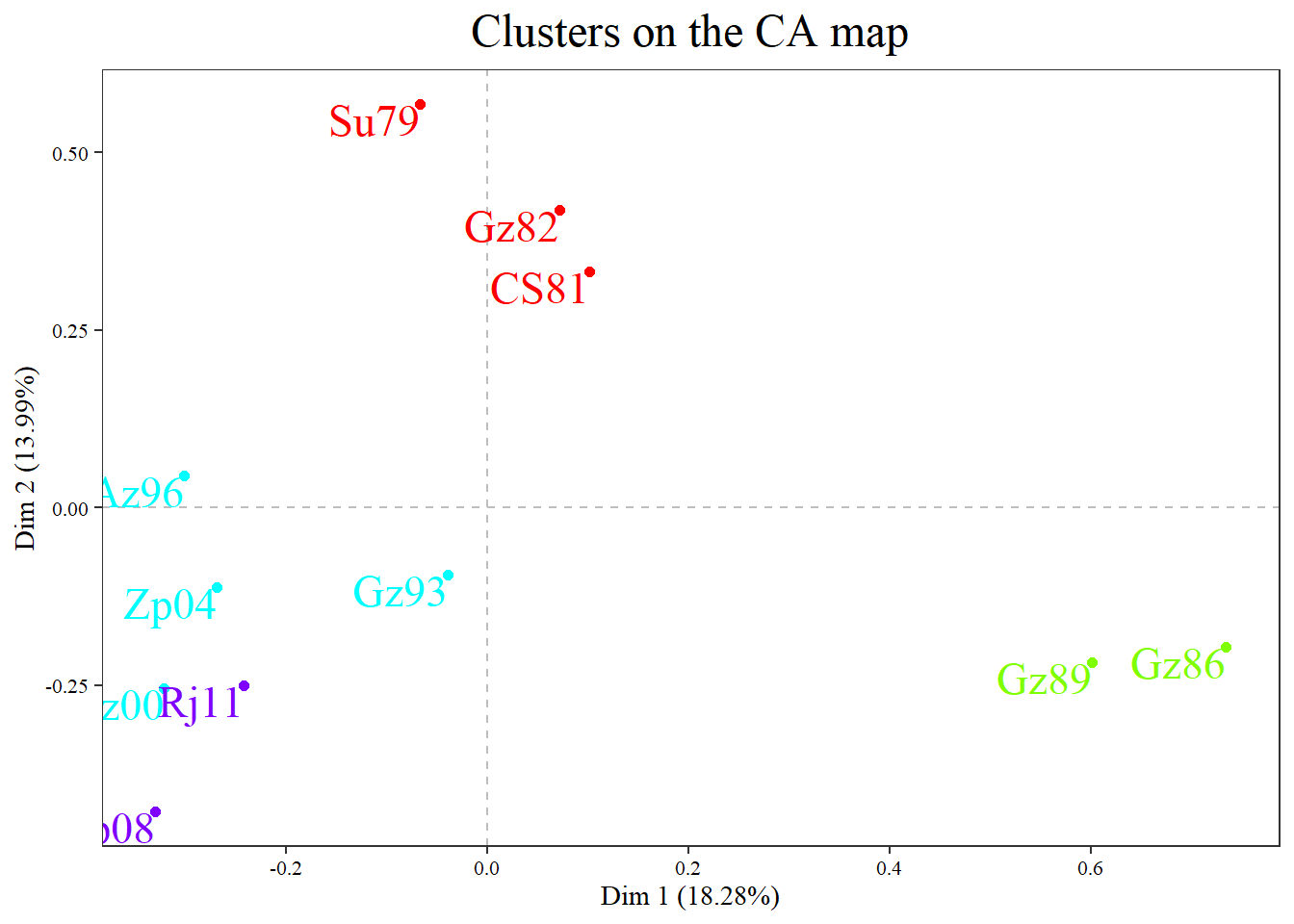###### plot.LexHCca(res.HCca , plot=c("points","labels"), labels=c(size=7,force=0, hjust="left", vjust="right"), xlim=c(-.5,.9))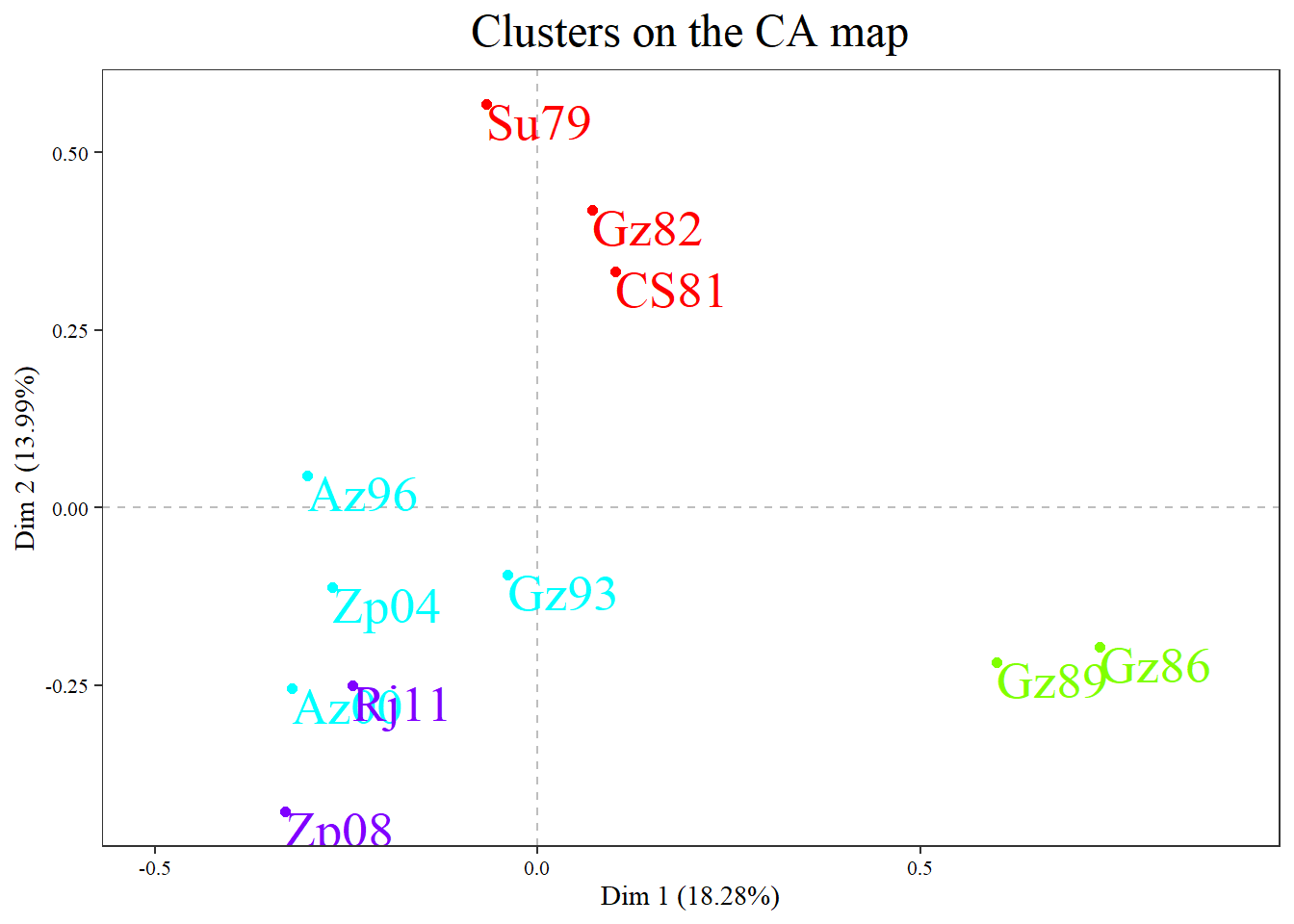###### plot.LexHCca(res.HCca, plot=c("labels"), labels=c(numbers=TRUE, size=6, family="serif", face="bold.italic"))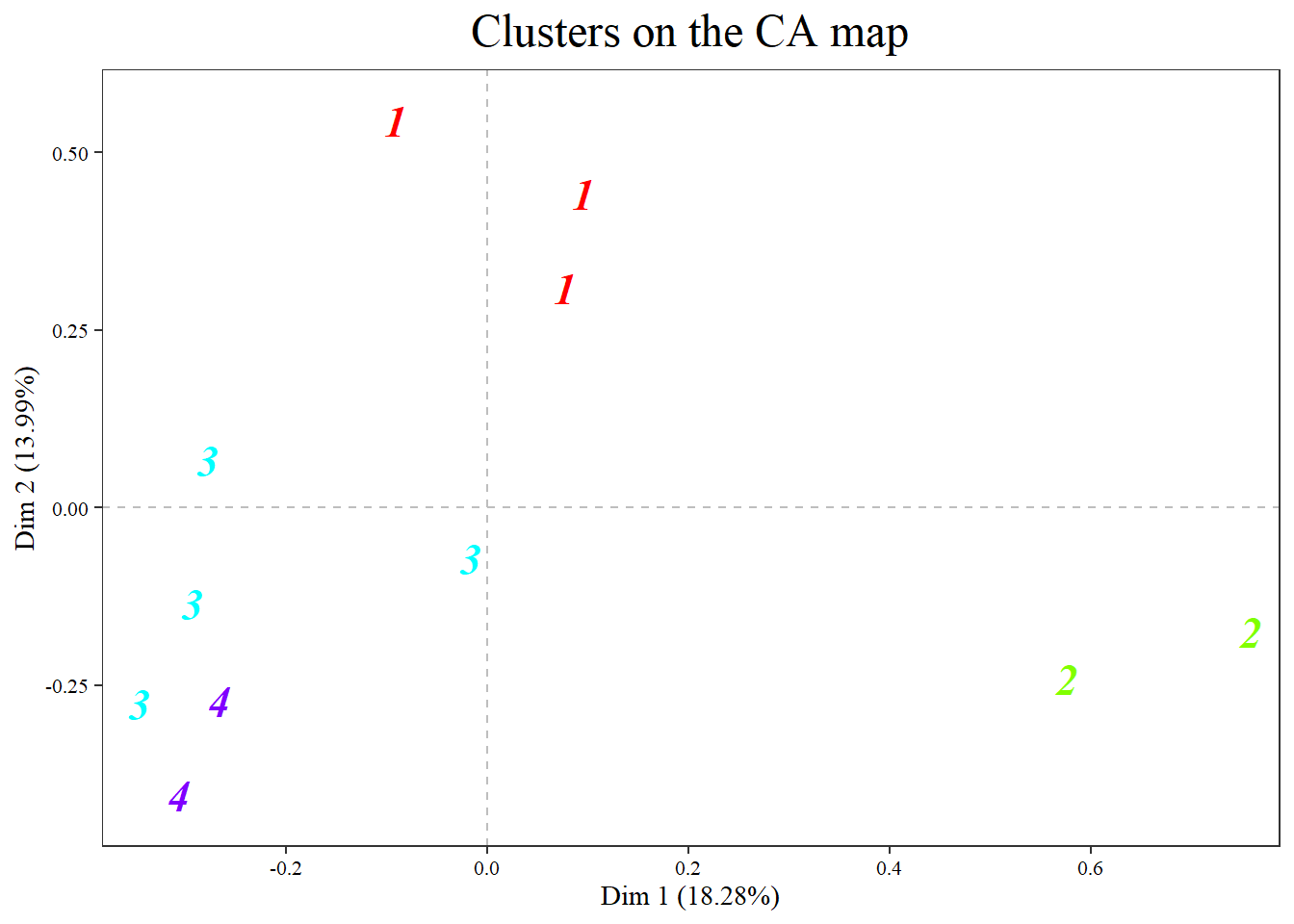###### plot.LexHCca(res.HCca, plot=c("points","labels"), labels=c(size=7,rect=TRUE))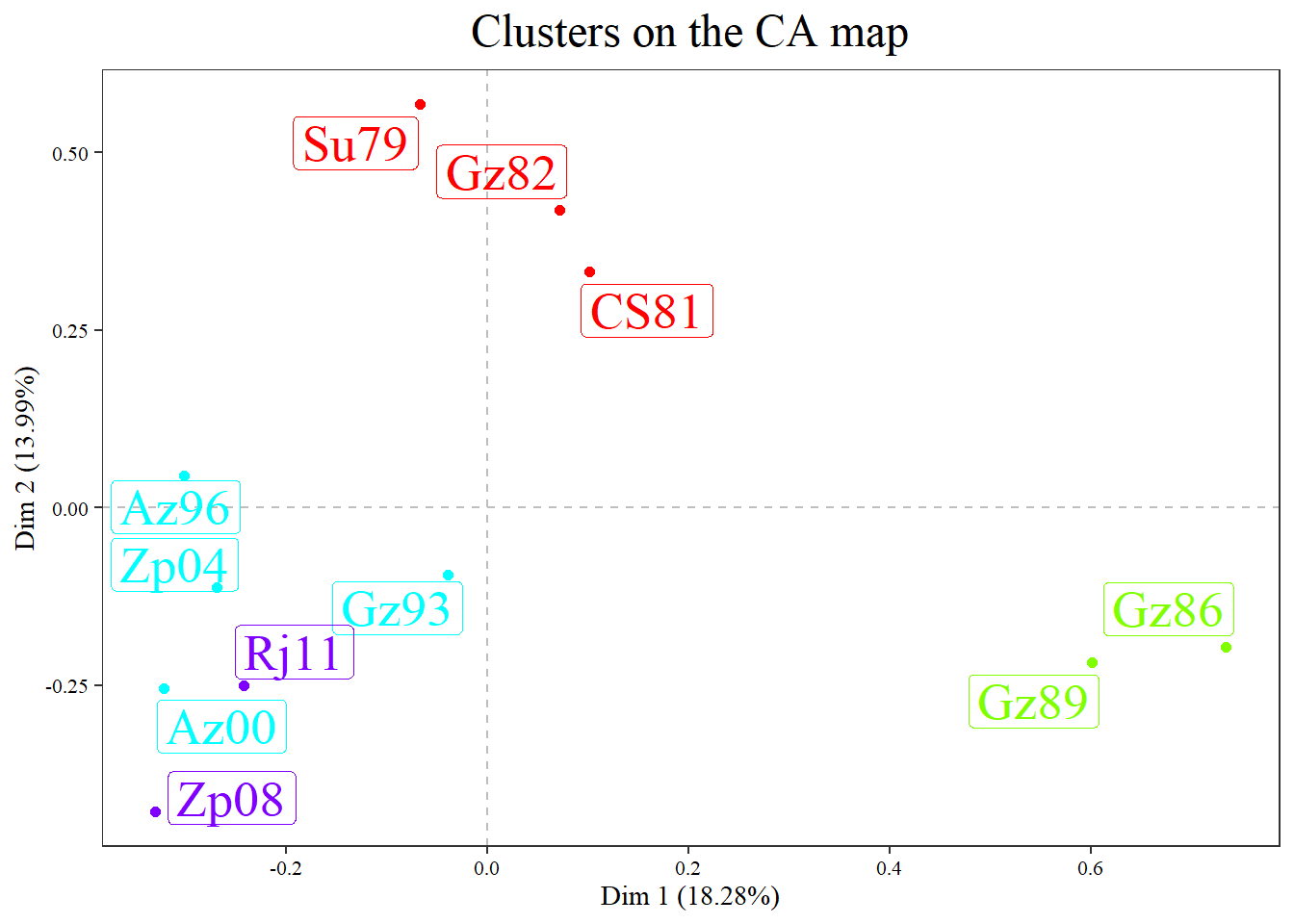###### plot.LexHCca(res.HCca, plot=c("points","labels"), labels=c(color.text="black",size=5,rect=TRUE))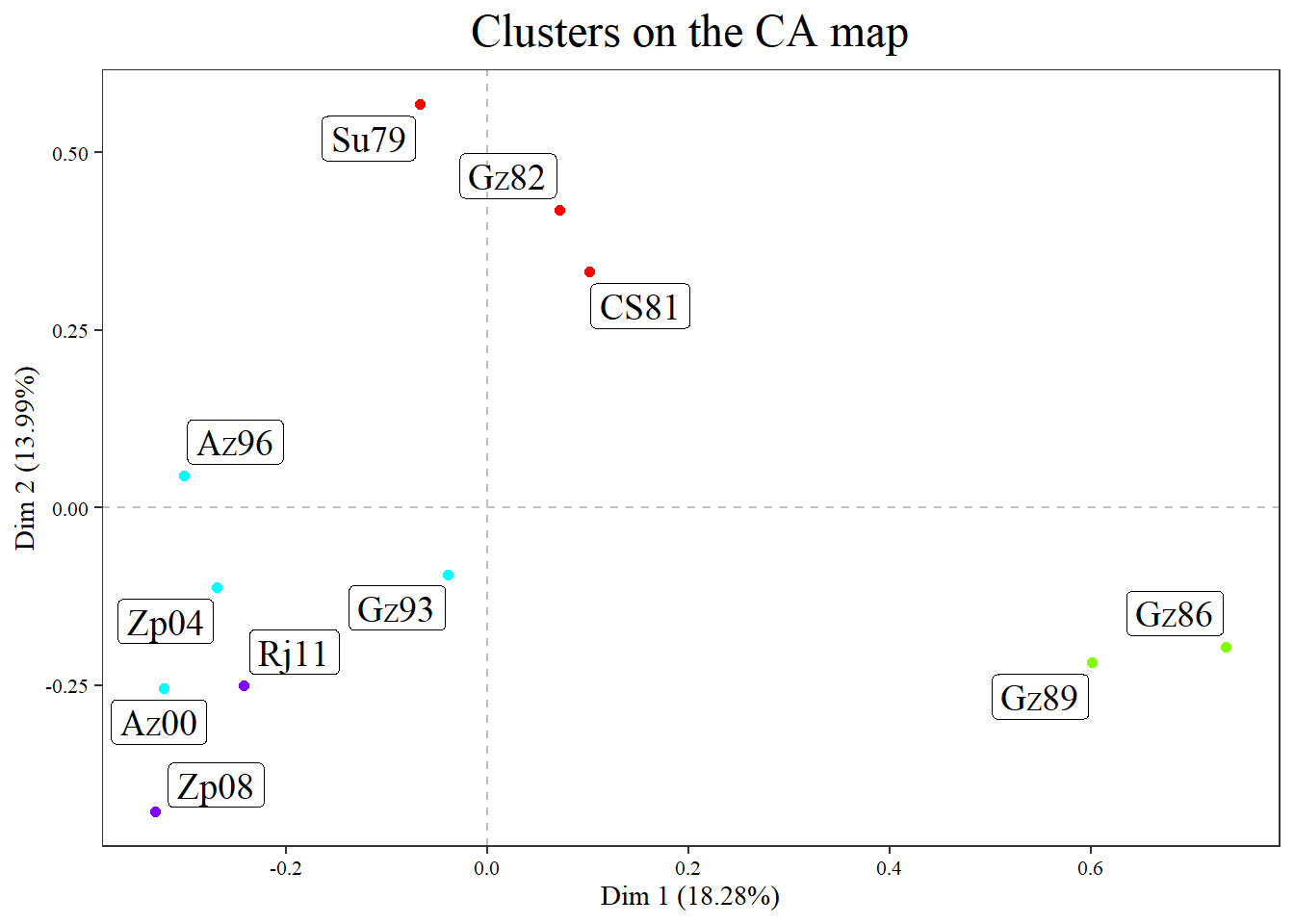###### plot.LexHCca(res.HCca, plot=c("labels"), palette=c("black","red", "forestgreen", "blue"), labels=c(size=5,rect=TRUE))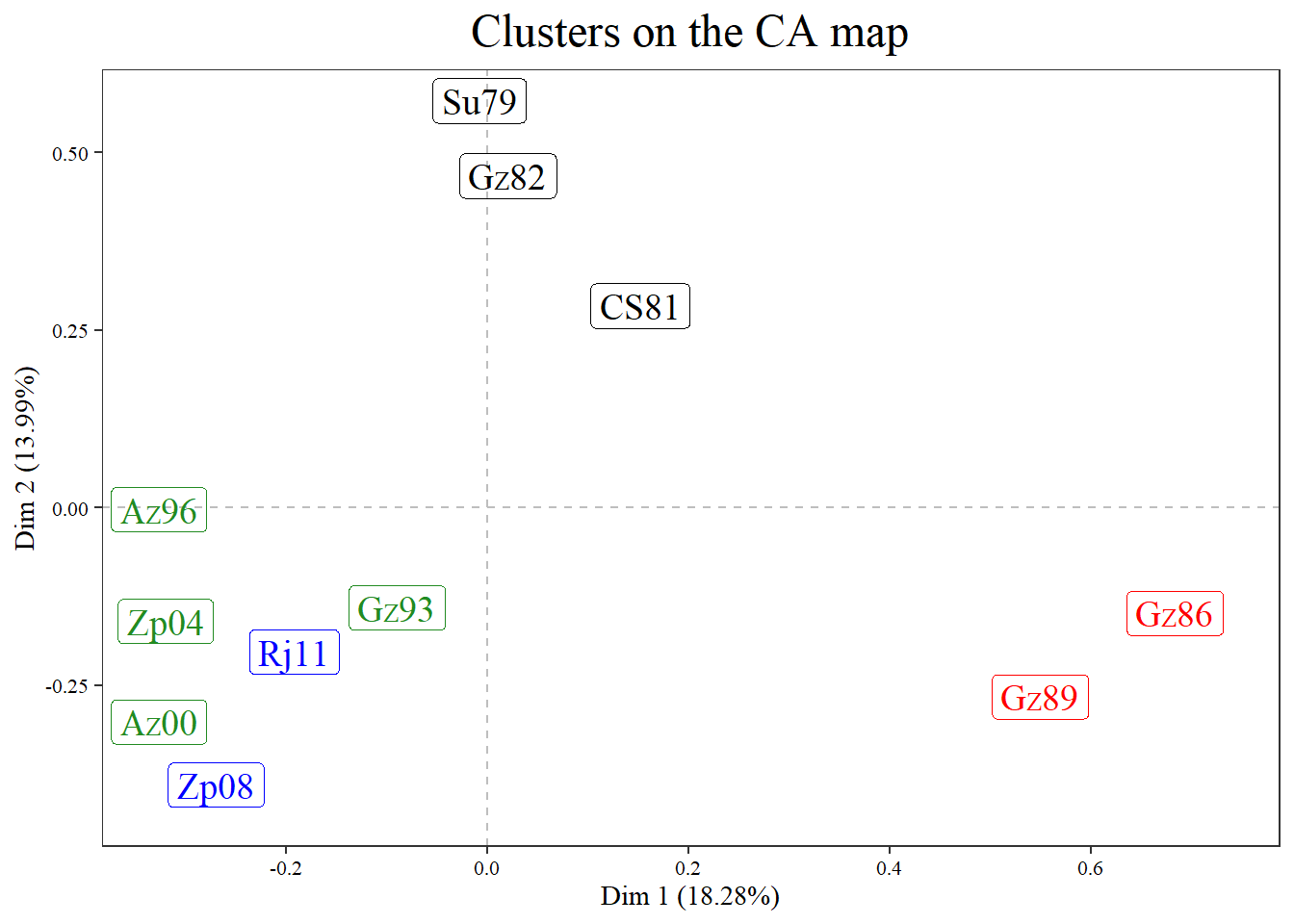Changing labels of the cases by numbers:

###### plot(res.HCca, type="map", plot=c("labels"), labels=c(numbers=TRUE))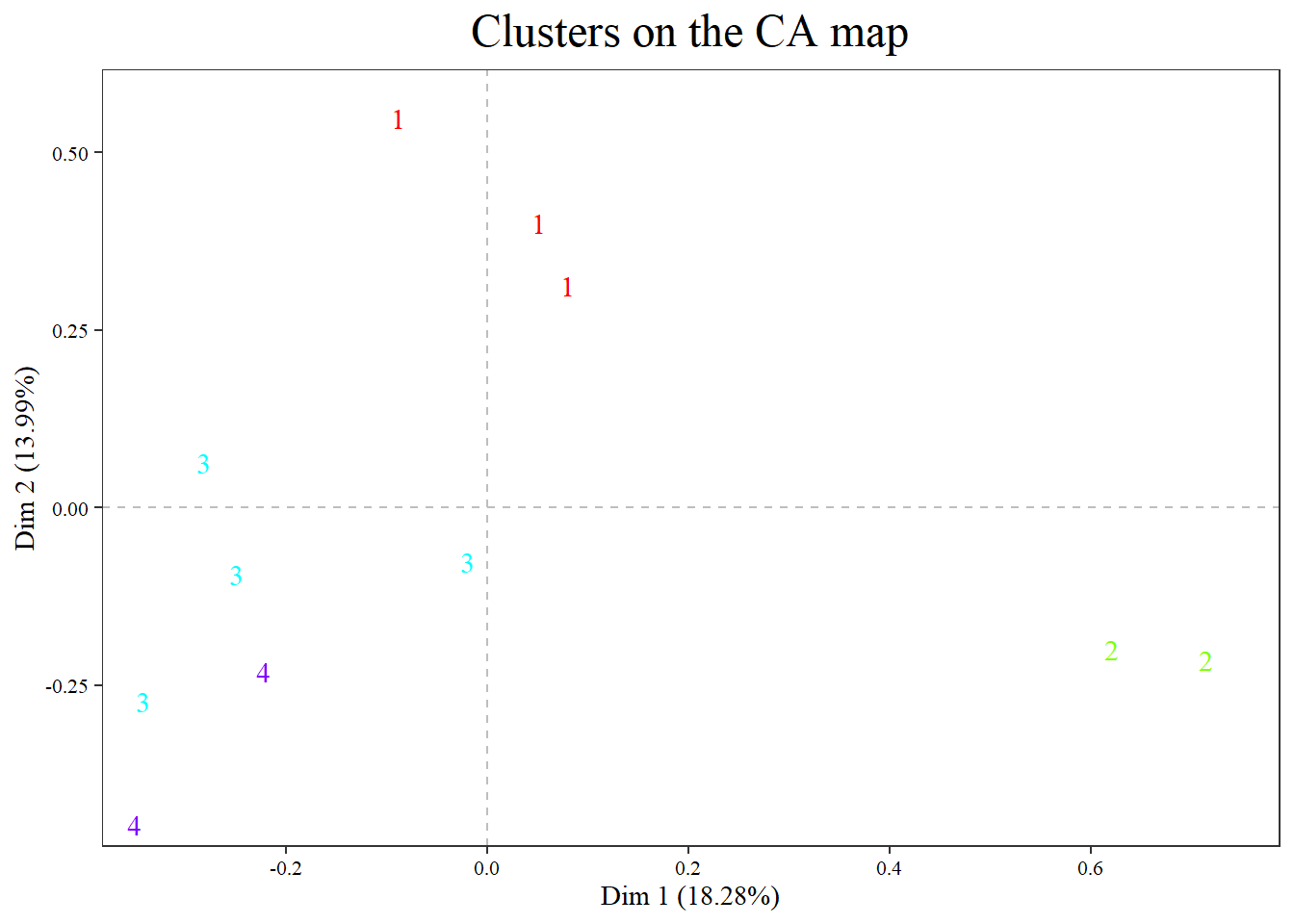### 5.7. Format of centers

It is possible manually change labels and colors:

###### plot.LexHCca(res.HCca, plot=c("points","labels", "centers"), centers=c(size=9, family="sans", face="italic", color="red", labels=c("A","B","C","D")))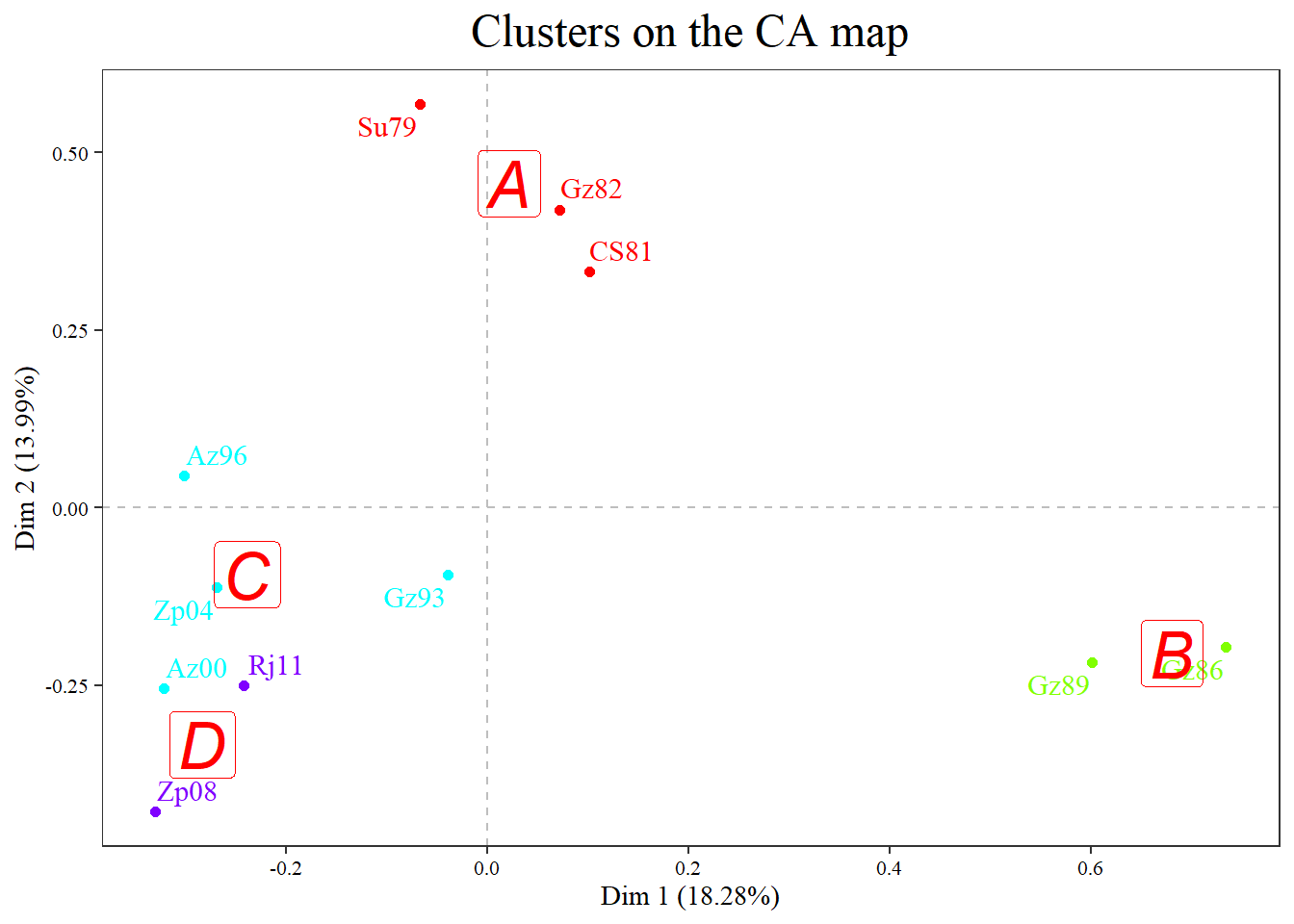###### plot.LexHCca(res.HCca, plot=c("points","labels", "centers"), centers=c(size=9, family="sans", face="italic", color="black", labels=c("A","B","C","D"),fill="TRUE"))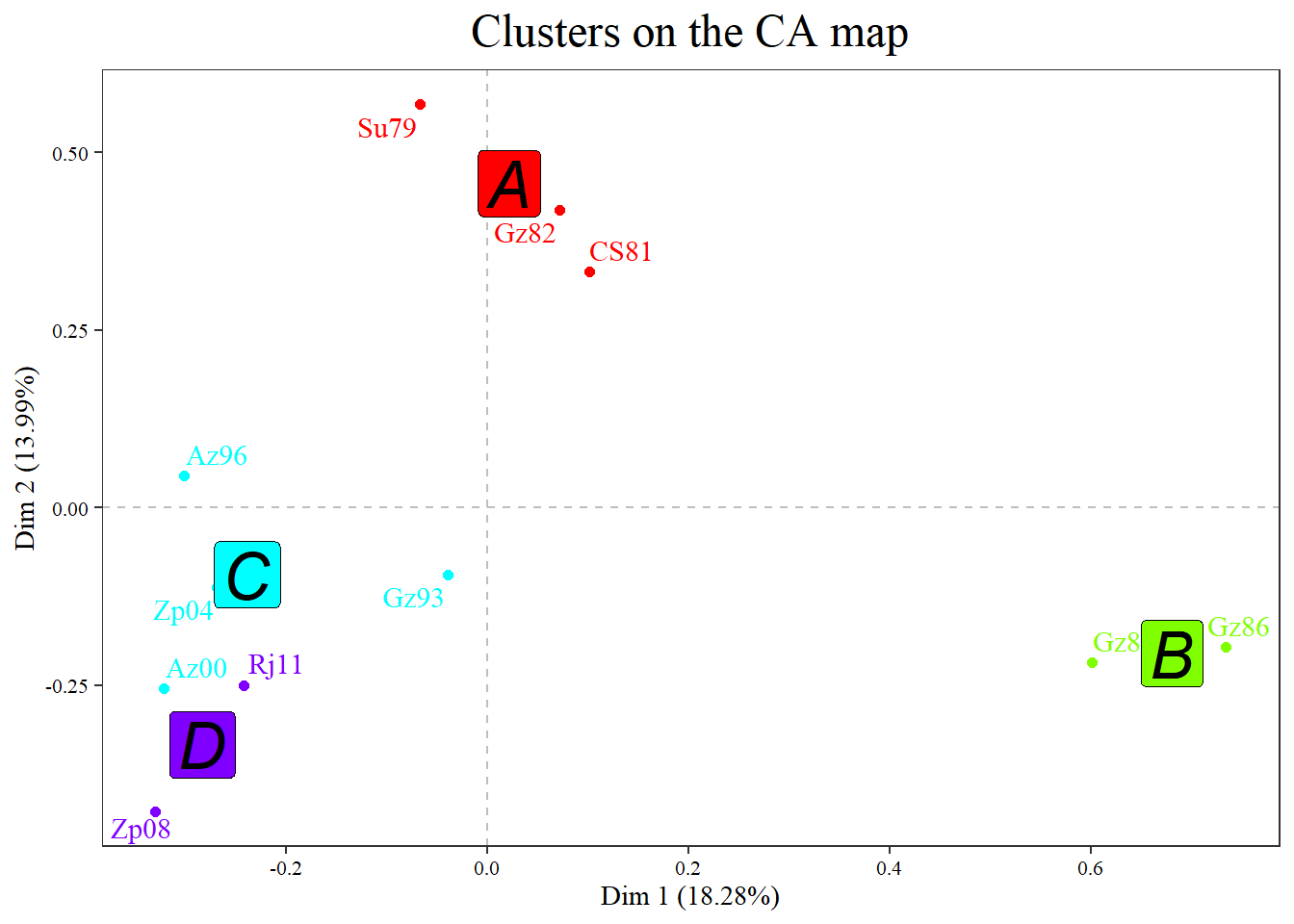###### plot.LexHCca(res.HCca, plot=c("points","labels", "centers"), centers=c(size=6, family="sans", face="italic", color="black", labels=c("A","B","C","D"),fill="yellow"))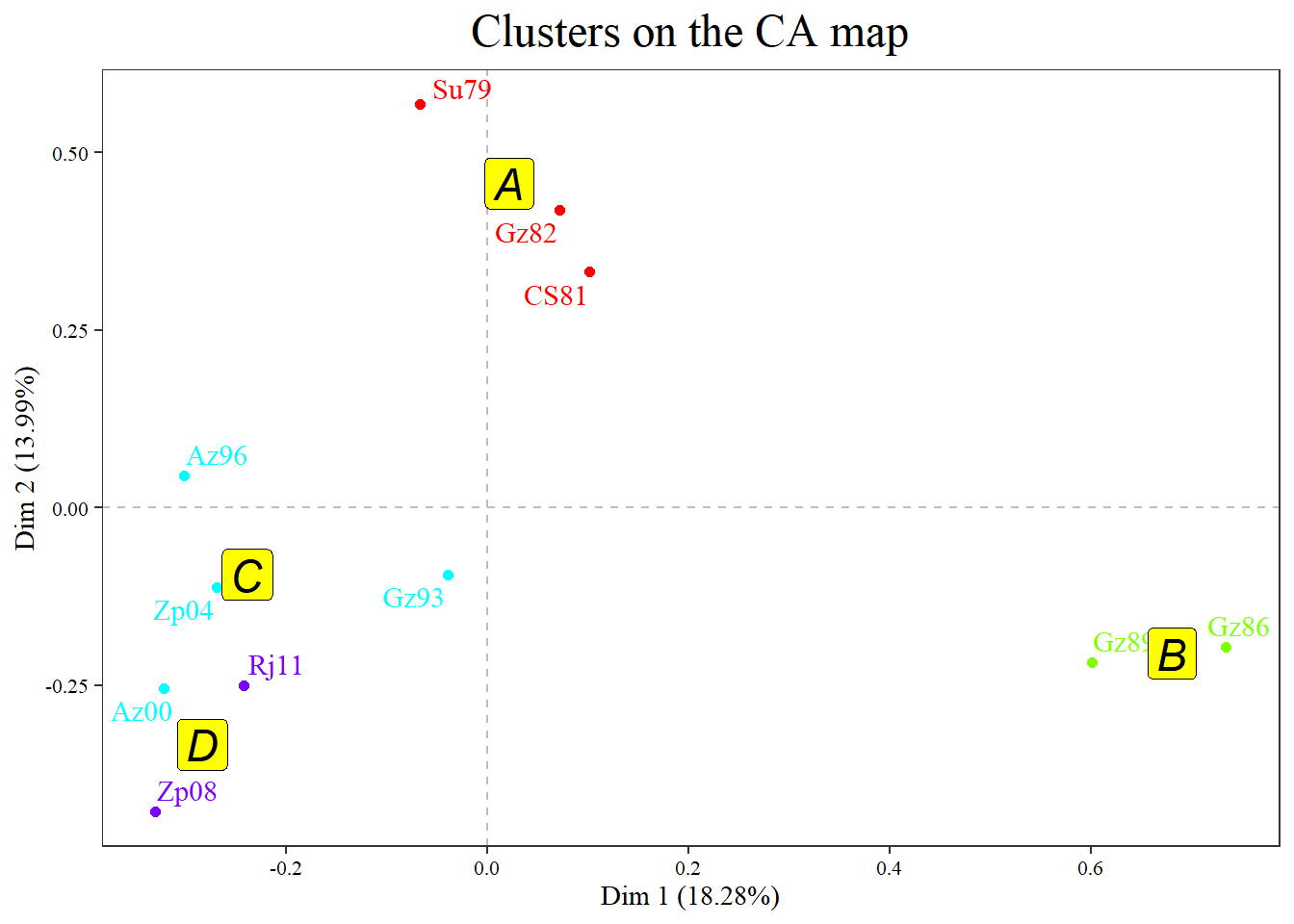###### plot.LexHCca(res.HCca, plot=c("points", "labels", "centers"), centers=c(size=4, family="sans", face="italic",fill=TRUE, color="black", alpha.t=0.2))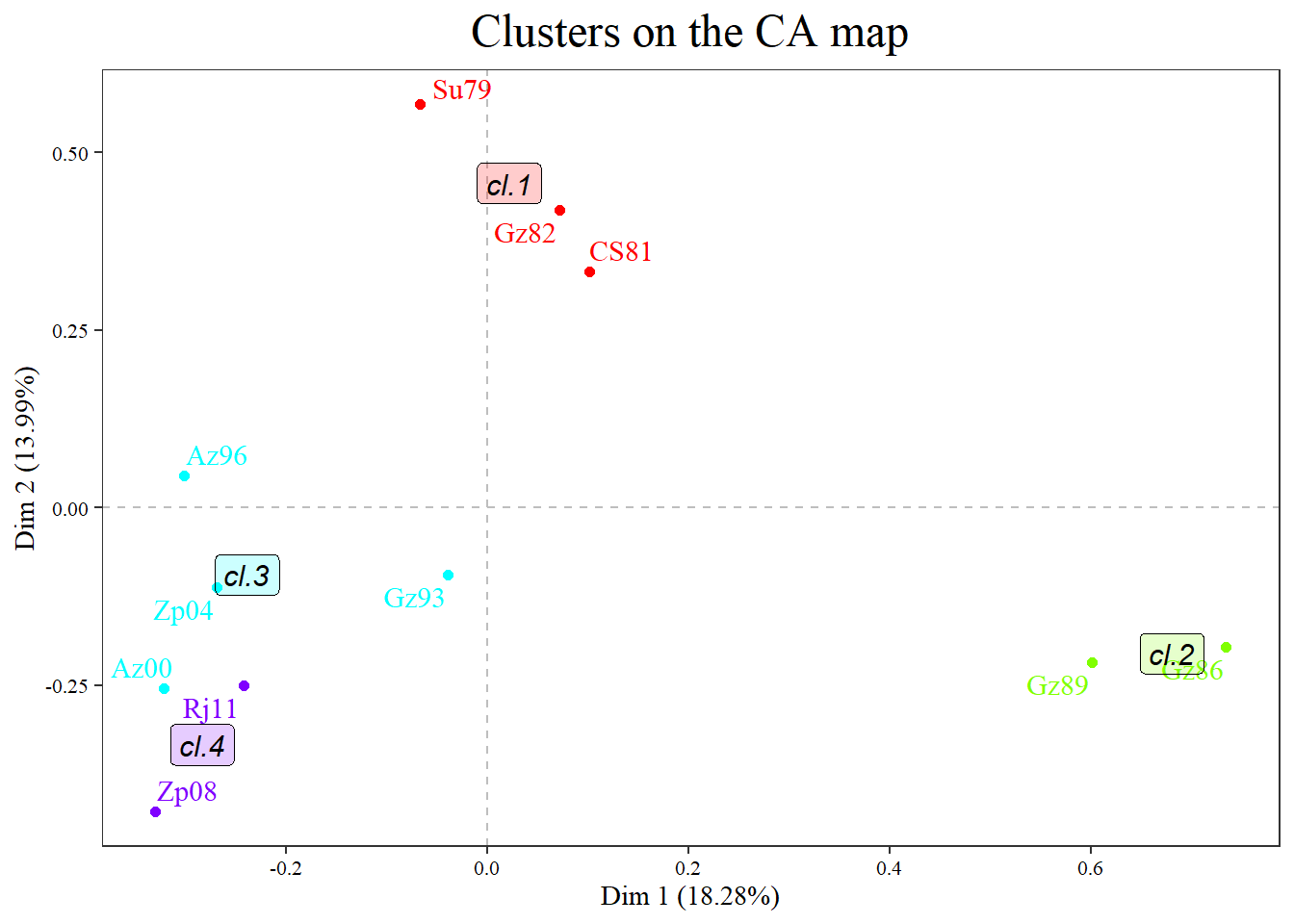###### plot.LexHCca(res.HCca, plot=c("points", "labels", "centers"), centers=c(size=5, family="sans", face="italic",fill=c("yellow"), color="red", alpha.t=0.2))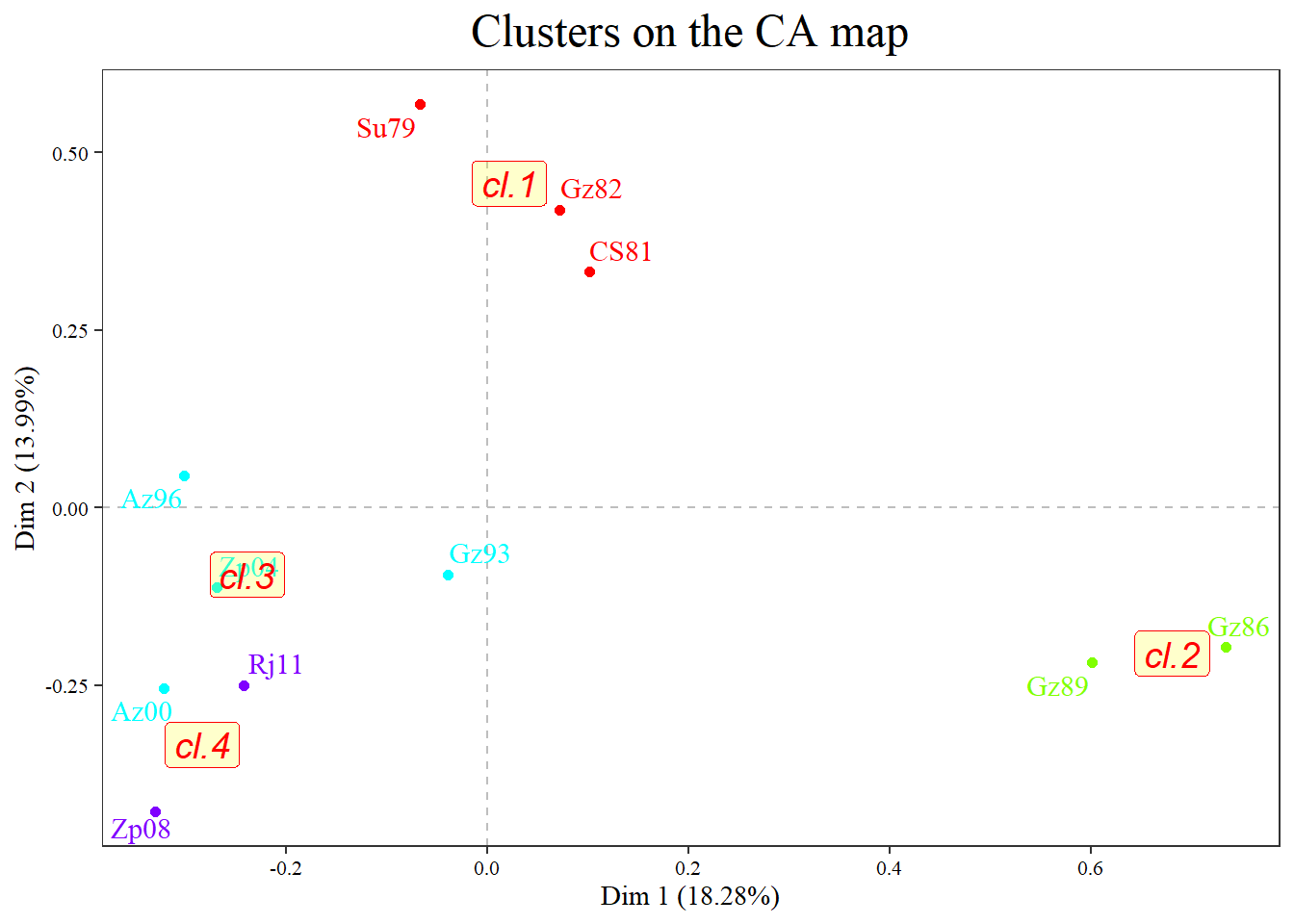### 5.8. Changing theme

###### plot.LexHCca(res.HCca, theme=theme_minimal())